# JP5665299B2 - Shift register circuit - Google Patents

## Info

Publication number
JP5665299B2
JP5665299B2 JP2009233035A JP2009233035A JP5665299B2 JP 5665299 B2 JP5665299 B2 JP 5665299B2 JP 2009233035 A JP2009233035 A JP 2009233035A JP 2009233035 A JP2009233035 A JP 2009233035A JP 5665299 B2 JP5665299 B2 JP 5665299B2
Authority
JP
Japan
Prior art keywords
node
transistor
shift register
circuit
level
Prior art date
Legal status (The legal status is an assumption and is not a legal conclusion. Google has not performed a legal analysis and makes no representation as to the accuracy of the status listed.)
Active
Application number
JP2009233035A
Other languages
Japanese (ja)
Other versions
JP2010135050A5 (en
JP2010135050A (en
Inventor

Original Assignee

Priority date (The priority date is an assumption and is not a legal conclusion. Google has not performed a legal analysis and makes no representation as to the accuracy of the date listed.)
Filing date
Publication date
Priority to JP2008280837 priority Critical
Priority to JP2008280837 priority
Application filed by 三菱電機株式会社 filed Critical 三菱電機株式会社
Priority to JP2009233035A priority patent/JP5665299B2/en
Publication of JP2010135050A publication Critical patent/JP2010135050A/en
Publication of JP2010135050A5 publication Critical patent/JP2010135050A5/ja
Application granted granted Critical
Publication of JP5665299B2 publication Critical patent/JP5665299B2/en
Active legal-status Critical Current
Anticipated expiration legal-status Critical

• 230000004913 activation Effects 0.000 claims description 60
• 239000003990 capacitor Substances 0.000 claims description 30
• 238000007599 discharging Methods 0.000 claims description 27
• 230000001808 coupling Effects 0.000 claims description 25
• 238000010168 coupling process Methods 0.000 claims description 25
• 238000005859 coupling reactions Methods 0.000 claims description 25
• 230000003071 parasitic Effects 0.000 claims description 16
• 230000001429 stepping Effects 0.000 claims 2
• 230000004048 modification Effects 0.000 description 107
• 238000006011 modification reactions Methods 0.000 description 107
• 238000010586 diagrams Methods 0.000 description 73
• 230000000875 corresponding Effects 0.000 description 26
• 230000001965 increased Effects 0.000 description 24
• 101710073810 25-Hydroxyvitamin D3 1-alpha-Hydroxylase Proteins 0.000 description 22
• 102100009529 25-hydroxyvitamin D-1 alpha hydroxylase, mitochondrial Human genes 0.000 description 22
• 230000000694 effects Effects 0.000 description 22
• 239000004973 liquid crystal related substances Substances 0.000 description 19
• 230000000630 rising Effects 0.000 description 17
• 239000000758 substrates Substances 0.000 description 16
• 230000003334 potential Effects 0.000 description 12
• 230000001629 suppression Effects 0.000 description 12
• 230000003111 delayed Effects 0.000 description 10
• 201000001195 sepiapterin reductase deficiency Diseases 0.000 description 8
• 230000000903 blocking Effects 0.000 description 6
• 239000004065 semiconductors Substances 0.000 description 6
• 238000000034 methods Methods 0.000 description 5
• 238000004519 manufacturing process Methods 0.000 description 4
• 230000000087 stabilizing Effects 0.000 description 4
• 230000001360 synchronised Effects 0.000 description 4
• 230000015572 biosynthetic process Effects 0.000 description 3
• 230000003247 decreasing Effects 0.000 description 3
• 238000005401 electroluminescence Methods 0.000 description 3
• 239000010408 films Substances 0.000 description 3
• 238000005755 formation reactions Methods 0.000 description 3
• 239000011521 glasses Substances 0.000 description 3
• 239000010410 layers Substances 0.000 description 3
• 102220440091 AMFR Q16D Human genes 0.000 description 2
• 208000004104 Gestational Diabetes Diseases 0.000 description 2
• 230000002457 bidirectional Effects 0.000 description 2
• 201000005569 gout Diseases 0.000 description 2
• 239000011159 matrix materials Substances 0.000 description 2
• 239000011347 resins Substances 0.000 description 2
• 229920005989 resins Polymers 0.000 description 2
• -1 SOI Substances 0.000 description 1
• 229910007541 Zn O Inorganic materials 0.000 description 1
• 230000003213 activating Effects 0.000 description 1
• 239000012190 activators Substances 0.000 description 1
• 229910021417 amorphous silicon Inorganic materials 0.000 description 1
• 239000003086 colorants Substances 0.000 description 1
• 230000000295 complement Effects 0.000 description 1
• 230000002950 deficient Effects 0.000 description 1
• 230000001934 delay Effects 0.000 description 1
• 230000002708 enhancing Effects 0.000 description 1
• 230000007274 generation of a signal involved in cell-cell signaling Effects 0.000 description 1
• 239000012212 insulators Substances 0.000 description 1
• 239000000463 materials Substances 0.000 description 1
• 229910021421 monocrystalline silicon Inorganic materials 0.000 description 1
• 229910021420 polycrystalline silicon Inorganic materials 0.000 description 1
• 229920005591 polysilicon Polymers 0.000 description 1
• 230000003252 repetitive Effects 0.000 description 1
• 239000010409 thin films Substances 0.000 description 1
• 230000001960 triggered Effects 0.000 description 1

## Images

••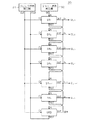•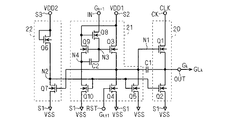•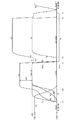•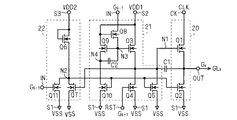•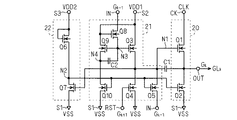•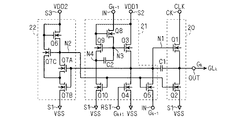•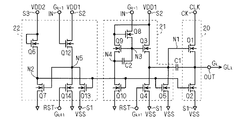•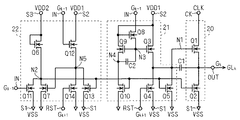•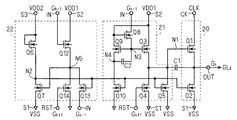•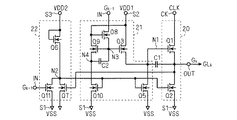•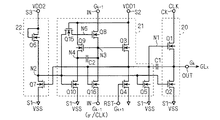•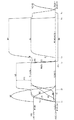•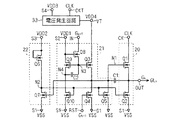•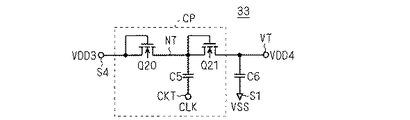•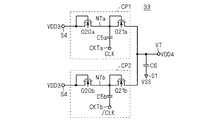•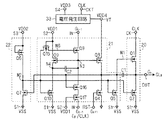•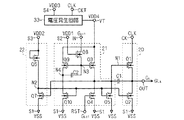•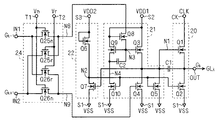•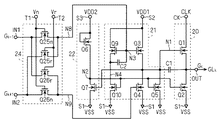•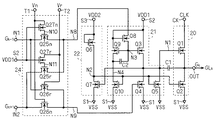•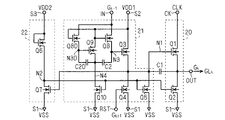•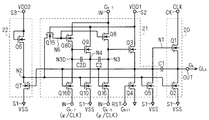•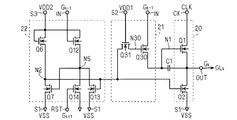•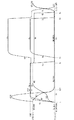•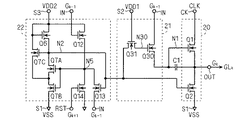•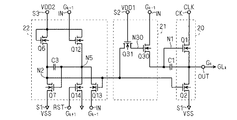•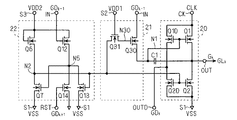•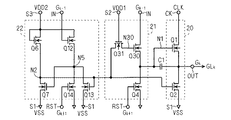•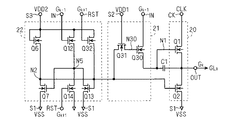•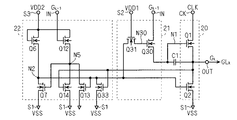•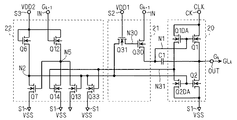•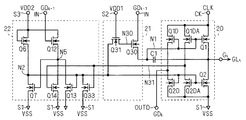•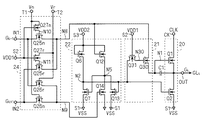•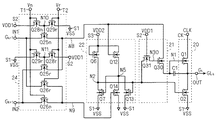•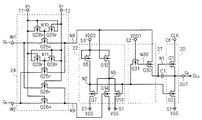•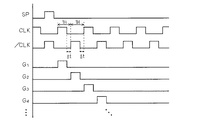•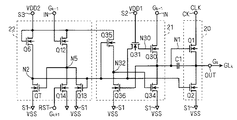•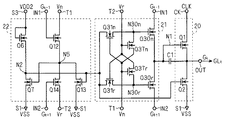•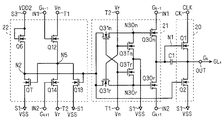## Classifications

• GPHYSICS
• G11INFORMATION STORAGE
• G11CSTATIC STORES
• G11C19/00Digital stores in which the information is moved stepwise, e.g. shift register stack stores, push-down stores
• G11C19/28Digital stores in which the information is moved stepwise, e.g. shift register stack stores, push-down stores using semiconductor elements
• GPHYSICS
• G11INFORMATION STORAGE
• G11CSTATIC STORES
• G11C19/00Digital stores in which the information is moved stepwise, e.g. shift register stack stores, push-down stores
• G11C19/18Digital stores in which the information is moved stepwise, e.g. shift register stack stores, push-down stores using capacitors as main elements of the stages
• G11C19/182Digital stores in which the information is moved stepwise, e.g. shift register stack stores, push-down stores using capacitors as main elements of the stages in combination with semiconductor elements, e.g. bipolar transistors, diodes
• G11C19/184Digital stores in which the information is moved stepwise, e.g. shift register stack stores, push-down stores using capacitors as main elements of the stages in combination with semiconductor elements, e.g. bipolar transistors, diodes with field-effect transistors, e.g. MOS-FET

## Description

BACKGROUND OF THE INVENTION 1. Field of the Invention The present invention relates to a scanning line driving circuit, and in particular, scanning line driving configured by using only field effect transistors of the same conductivity type used in electro-optical devices such as image display devices and image sensors. It relates to the circuit.

In an image display device such as a liquid crystal display device (hereinafter “display device”), a gate line (scanning line) is provided for each pixel row (pixel line) of a display panel in which a plurality of pixels are arranged in a matrix, and a display signal is displayed. The display image is updated by sequentially selecting and driving the gate lines in the period of one horizontal period. As such a gate line driving circuit (scanning line driving circuit) for sequentially selecting and driving pixel lines, that is, gate lines, a shift register that performs a shift operation that makes a round in one frame period of a display signal can be used. .

The shift register used in the gate line driver circuit is preferably composed of only field effect transistors of the same conductivity type in order to reduce the number of steps in the manufacturing process of the display device. For this reason, various shift registers composed of only N-type or P-type field effect transistors and display devices equipped with the shift registers have been proposed (for example, Patent Document 1).

JP 2004-78172 A

A shift register as a gate line driving circuit is configured by cascading a plurality of shift register circuits provided for each pixel line, that is, for each gate line. In this specification, for convenience of explanation, each of the plurality of shift register circuits constituting the gate line driving circuit is referred to as a “unit shift register”. That is, the output terminal of each unit shift register constituting the gate line driving circuit is connected to the input terminal of the next stage or subsequent unit shift register.

FIG. 7 of Patent Document 1 shows the configuration of a conventional unit shift register. As shown in the figure, the conventional unit shift register includes a first transistor (M1) connected between an output terminal (GOUT [N]) and a clock terminal (CKV), an output terminal, and a first transistor. And a second transistor (M2) connected to the power supply terminal (VOFF). The output signal of the unit shift register is output by transmitting a clock signal input to the clock terminal to the output terminal in a state where the first transistor is on and the second transistor is off.

Particularly, since the gate line driving circuit needs to be activated by charging the gate line at high speed using the output signal, in each unit shift register constituting the gate line driving circuit, the first transistor has a high driving capability (current is supplied). Flow ability) is required. Therefore, it is desirable that the gate-source voltage be maintained in a high state while the first transistor is turned on.

A third transistor (M3) for charging the first node is connected to the first node (N1) to which the gate of the first transistor is connected. In the conventional unit shift register, the third transistor is connected between the first node and the second power supply terminal (VON), and its gate is the input terminal of the unit shift register (that is, the output terminal of the previous unit shift register ( GOUT [N-1])). That is, the third transistor is turned on when the output signal of the unit shift register in the previous stage is activated, and charges are supplied from the power supply connected to the second power supply terminal to the first node to charge the first node (precharge). To do. Thereby, the first transistor is turned on, and when the clock signal subsequently becomes high (H) level, it is transmitted to the output terminal and an output signal is output.

In the shift register circuit of Patent Document 1, a capacitive element (C) is provided between the output terminal, that is, the source of the first transistor and the first node. Therefore, when the first transistor is turned on by the precharge of the first node and the output terminal subsequently becomes H level in response to the clock signal, the first node is boosted by the coupling through the capacitor element, and the first transistor The gate-source voltage is kept high. As a result, the first transistor has a high driving capability.

However, the voltage between the gate and the source of the first transistor while the first node is boosted does not increase from the state before boosting, but is only kept substantially the same. In other words, the driving capability of the first transistor in the unit shift register is determined by the gate-source voltage given at the time of precharging by the third transistor. That is, in order to increase the drive capability of the first transistor, it is necessary to charge the first node to a sufficiently high level at the precharge stage.

When the potential of the second power supply terminal and the H level of the clock signal are VDD, and the threshold voltage of the third transistor is Vth, the potential of the first node theoretically rises to VDD−Vth by precharging. However, when the frequency of the clock signal is increased and the pulse width of the input signal (the output signal of the previous unit shift register) is narrowed, it is difficult to reach the first node to the maximum precharge level (VDD−Vth). become. The reason is that the third transistor (M3) operates in the source follower mode at the time of precharging the first node. That is, since the gate-source voltage of the third transistor decreases as the level of the first node increases, the driving capability of the third transistor decreases as the charging of the first node proceeds, and the level increase speed increases. It is because it falls.

That is, in the conventional unit shift register, since the gate (first node) of the first transistor is precharged by the third transistor operating in the source follower mode, the first node is charged to the maximum precharge level. It took a relatively long time. For this reason, when the frequency of the clock signal is increased, the first node cannot be sufficiently precharged, resulting in a decrease in the driving capability of the first transistor. In particular, the gate line driving circuit is problematic because it is necessary to charge and activate the gate line at high speed using the output signal of the unit shift register, and the high driving capability is required for the first transistor. That is, since it is difficult to increase the operation speed of the gate line driving circuit by increasing the frequency of the clock signal, there arises a problem that the resolution of the display device is hindered.

The present invention has been made to solve the above-described problems, and an object of the present invention is to provide a shift register circuit capable of suppressing a decrease in driving capability when the frequency of a clock signal increases.

The shift register circuit according to the present invention includes an input terminal, an output terminal, a clock terminal, a first transistor that supplies a clock signal input to the clock terminal to the output terminal, and a second transistor that discharges the output terminal. A charge-discharge of a first node connected to the control electrode of the first transistor, and a charge-discharge of a second node connected to the control electrode of the second transistor; And a pull-down driving circuit that drives the second transistor by performing the operation of the first node according to activation of an input signal input to the input terminal. The third transistor for charging the battery and the control electrode of the third transistor are connected in response to the activation of the input signal Comprises three nodes the third transistor and a boosting means for boosting to a level that operates in the unsaturated region, the third transistor is connected between the input terminal and the first node, said boosting means Includes a charge / discharge circuit that charges the third node prior to the activation of the input signal and discharges the third node prior to the deactivation of the input signal. a it shall be performed by the parasitic capacitance of the third transistor.

According to the shift register circuit of the present invention, according to the shift register circuit of the present invention, the third transistor operates in the non-saturation region to charge the first node (precharge). Therefore, even when the frequency of the clock signal is increased and the pulse width of the signal input to the input terminal is reduced, the first node can be sufficiently precharged. That is, it is possible to prevent a decrease in the driving capability of the first transistor. In addition, since the third transistor operates in the non-saturated region, a loss corresponding to the threshold voltage does not occur, and the first node can be precharged to a higher level than in the prior art. The driving ability is increased.

It is a schematic block diagram which shows the structure of a liquid crystal display device. 1 is a diagram illustrating a configuration of a gate line driving circuit according to a first embodiment. FIG. 3 is a circuit diagram of a unit shift register according to the first embodiment. FIG. 5 is a timing diagram for explaining the operation of the unit shift register according to the first embodiment. 10 is a circuit diagram of a unit shift register according to a second modification of the first embodiment. FIG. FIG. 10 is a circuit diagram of a unit shift register according to a third modification of the first embodiment. FIG. 10 is a circuit diagram of a unit shift register according to a fourth modification example of the first embodiment. FIG. 10 is a circuit diagram of a unit shift register according to a fifth modification example of the first embodiment. FIG. 10 is a circuit diagram of a unit shift register according to a fifth modification example of the first embodiment. FIG. 10 is a circuit diagram of a unit shift register according to a fifth modification example of the first embodiment. FIG. 10 is a circuit diagram of a unit shift register according to a sixth modification of the first embodiment. 6 is a circuit diagram of a unit shift register according to Embodiment 2. FIG. FIG. 10 is a timing diagram for explaining the operation of the unit shift register according to the second embodiment. FIG. 6 is a circuit diagram of a unit shift register according to a third embodiment. FIG. 6 is a circuit diagram of a voltage generation circuit according to a third embodiment. FIG. 10 is a circuit diagram showing a configuration of a voltage generation circuit according to a first modification of the third embodiment. FIG. 10 is a circuit diagram of a unit shift register according to a second modification example of the third embodiment. FIG. 15 is a circuit diagram of a unit shift register according to a third modification example of the third embodiment. FIG. 6 is a circuit diagram of a unit shift register according to a fourth embodiment. FIG. 16 is a circuit diagram of a unit shift register according to a first modification example of the fourth embodiment. FIG. 16 is a circuit diagram of a unit shift register according to a second modification example of the fourth embodiment. FIG. 10 is a circuit diagram of a unit shift register according to a fifth embodiment. FIG. 10 is a circuit diagram of a unit shift register according to a modification of the fifth embodiment. FIG. 10 is a circuit diagram of a unit shift register according to a sixth embodiment. FIG. 16 is a timing diagram for explaining the operation of a unit shift register according to the sixth embodiment. FIG. 29 is a circuit diagram of a unit shift register according to a third modification example of the sixth embodiment. FIG. 29 is a circuit diagram of a unit shift register according to a fourth modification example of the sixth embodiment. FIG. 29 is a circuit diagram of a unit shift register according to a fifth modification example of the sixth embodiment. FIG. 29 is a circuit diagram of a unit shift register according to a sixth modification example of the sixth embodiment. FIG. 29 is a circuit diagram of a unit shift register according to a seventh modification example of the sixth embodiment. FIG. 29 is a circuit diagram of a unit shift register according to an eighth modification example of the sixth embodiment. FIG. 29 is a circuit diagram of a unit shift register according to a ninth modification example of the sixth embodiment. FIG. 38 is a circuit diagram of a unit shift register according to a tenth modification example of the sixth embodiment. FIG. 10 is a circuit diagram of a unit shift register according to a seventh embodiment. FIG. 32 is a circuit diagram of a unit shift register according to a first modification example of the seventh embodiment. FIG. 32 is a circuit diagram of a unit shift register according to a second modification example of the seventh embodiment. It is a signal waveform diagram showing the operation of the gate line drive circuit. FIG. 38 is a circuit diagram of a unit shift register according to an eleventh modification of the sixth embodiment. FIG. 10 is a circuit diagram of a unit shift register according to an eighth embodiment. FIG. 29 is a circuit diagram of a unit shift register according to a modification of the eighth embodiment.

Embodiments of the present invention will be described below with reference to the drawings. In addition, in order to avoid duplication and redundant description, elements having the same or corresponding functions are denoted by the same reference symbols in the respective drawings.

The transistor used in each embodiment is an insulated gate field effect transistor. In the insulated gate field effect transistor, the electric conductivity between the drain region and the source region in the semiconductor layer is controlled by the electric field in the gate insulating film. As a material of the semiconductor layer in which the drain region and the source region are formed, an organic semiconductor such as polysilicon, amorphous silicon, or pentacene, an oxide semiconductor such as single crystal silicon or IGZO (In-Ga-Zn-O), or the like is used. be able to.

As is well known, each transistor has a control electrode (gate (electrode) in the narrow sense), one current electrode (drain (electrode) or source (electrode) in the narrow sense)), and the other current electrode (in the narrow sense, the drain (electrode) or the source (electrode)). In a narrow sense, it is an element having at least three electrodes including a source (electrode) or a drain (electrode). The transistor functions as a switching element in which a channel is formed between the drain and the source by applying a predetermined voltage to the gate. The drain and source of the transistor have basically the same structure, and their names are interchanged depending on the applied voltage condition. For example, in the case of an N-type transistor, an electrode having a relatively high potential (hereinafter also referred to as “level”) is called a drain, and a low electrode is called a source (in the case of a P-type transistor, the opposite is true).

Unless otherwise specified, these transistors may be formed on a semiconductor substrate, or may be a thin film transistor (TFT) formed on an insulating substrate such as glass. The substrate over which the transistor is formed may be a single crystal substrate or an insulating substrate such as SOI, glass, or resin.

The gate line driving circuit of the present invention is configured using only a single conductivity type transistor. For example, an N-type transistor becomes active (on state, conductive state) when the gate-source voltage becomes H (high) level higher than the threshold voltage of the transistor, and L ( At a low level, it becomes inactive (off state, non-conductive state). Therefore, in a circuit using an N-type transistor, the H level of the signal is “active level” and the L level is “inactive level”. In addition, each node of a circuit configured using an N-type transistor is charged to become an H level, thereby causing a change from an inactive level to an active level, and being discharged to an L level. A change from to inactive level occurs.

Conversely, a P-type transistor becomes active (on state, conductive state) when the gate-source voltage becomes L level lower than the threshold voltage of the transistor (a negative value with respect to the source). It becomes inactive (OFF state, non-conducting state) at an H level higher than the voltage. Therefore, in a circuit using a P-type transistor, the L level of the signal is “active level” and the H level is “inactive level”. In addition, each node of the circuit configured using the P-type transistor has a charge / discharge relationship opposite to that in the case of the N-type transistor, and is charged to the L level, so that the inactive level changes from the inactive level to the active level. When the change occurs and is discharged to the H level, a change from the active level to the inactive level occurs.

In this specification, “connection” between two elements, between two nodes, or between one element and one node is a connection through other elements (elements, switches, etc.). In the following description, it is assumed to include a state that is substantially equivalent to a direct connection. For example, even if two elements are connected via a switch, if they can function in the same way as when they are directly connected, the two elements are “connected”. Express.

In the present invention, clock signals having different phases (multiphase clock signals) are used. In the following, for the sake of simplicity, a certain interval is provided between the active period of one clock signal and the active period of the clock signal to be activated next (Δt in FIG. 37). However, in the present invention, it is sufficient that the active periods of the clock signals do not substantially overlap, and the above-described interval may not be provided. For example, if the activation level is H level, the falling timing of one clock signal and the rising timing of the clock signal to be activated next may be simultaneous.

<Embodiment 1>
FIG. 1 is a schematic block diagram showing a configuration of a display device according to Embodiment 1 of the present invention, and shows an overall configuration of a liquid crystal display device 100 as a representative example of the display device. Note that the gate line driving circuit of the present invention is not limited to application to liquid crystal display devices, but is widely applied to electro-optical devices such as electroluminescence (EL), organic EL, plasma display, electronic paper, and image sensors. Is possible.

The liquid crystal display device 100 includes a liquid crystal array unit 10, a gate line driving circuit (scanning line driving circuit) 30, and a source driver 40. As will be apparent from the following description, the shift register according to the embodiment of the present invention is mounted on the gate line driving circuit 30.

The liquid crystal array unit 10 includes a plurality of pixels 15 arranged in a matrix. Each of the pixel rows (hereinafter also referred to as “pixel lines”) is provided with gate lines GL 1 , GL 2 ... (Generically referred to as “gate lines GL”), and each pixel row (hereinafter also referred to as “pixel column”). Are respectively provided with data lines DL 1 , DL 2 ... (Generic name “data line DL”). FIG. 1 representatively shows the pixels 15 in the first and second columns of the first row, and the corresponding gate lines GL 1 and data lines DL 1 and DL 2 .

Each pixel 15 includes a pixel switch element 16 provided between the corresponding data line DL and the pixel node Np, a capacitor 17 and a liquid crystal display element 18 connected in parallel between the pixel node Np and the common electrode node NC. have. The orientation of the liquid crystal in the liquid crystal display element 18 changes according to the voltage difference between the pixel node Np and the common electrode node NC, and the display brightness of the liquid crystal display element 18 changes in response to this. Thereby, the luminance of each pixel can be controlled by the display voltage transmitted to the pixel node Np via the data line DL and the pixel switch element 16. That is, by applying an intermediate voltage difference between the voltage difference corresponding to the maximum luminance and the voltage difference corresponding to the minimum luminance between the pixel node Np and the common electrode node NC, the intermediate luminance is reduced. Can be obtained. Therefore, gradation brightness can be obtained by setting the display voltage stepwise.

The gate line driving circuit 30 sequentially selects and activates the gate lines GL based on a predetermined scanning cycle. The gate electrodes of the pixel switch elements 16 are connected to the corresponding gate lines GL. While the specific gate line GL is selected, the pixel switch element 16 is in a conductive state in each pixel connected thereto, and the pixel node Np is connected to the corresponding data line DL. The display voltage transmitted to the pixel node Np is held by the capacitor 17. In general, the pixel switch element 16 is composed of a TFT formed on the same insulator substrate (glass substrate, resin substrate, etc.) as the liquid crystal display element 18.

The source driver 40 is for outputting a display voltage, which is set stepwise by a display signal SIG that is an N-bit digital signal, to the data line DL. Here, as an example, the display signal SIG is a 6-bit signal and is composed of display signal bits DB0 to DB5. Based on the 6-bit display signal SIG, 2 6 = 64 gradation display is possible in each pixel. Furthermore, if one color display unit is formed by three pixels of R (Red), G (Green), and B (Blue), approximately 260,000 colors can be displayed.

As shown in FIG. 1, the source driver 40 includes a shift register 50, data latch circuits 52 and 54, a gradation voltage generation circuit 60, a decode circuit 70, and an analog amplifier 80.

In the display signal SIG, display signal bits DB0 to DB5 corresponding to the display luminance of each pixel 15 are serially generated. In other words, the display signal bits DB0 to DB5 at each timing indicate the display luminance in any one pixel 15 in the liquid crystal array unit 10.

The shift register 50 instructs the data latch circuit 52 to take in the display signal bits DB0 to DB5 at a timing synchronized with a cycle in which the setting of the display signal SIG is switched. The data latch circuit 52 sequentially takes in the serially generated display signal SIG and holds the display signal SIG for one pixel line.

The latch signal LT input to the data latch circuit 54 is activated at the timing when the display signal SIG for one pixel line is taken into the data latch circuit 52. In response thereto, the data latch circuit 54 takes in the display signal SIG for one pixel line held in the data latch circuit 52 at that time.

The gradation voltage generation circuit 60 is composed of 63 voltage dividing resistors connected in series between the high voltage VDH and the low voltage VDL, and generates 64 gradation voltages V1 to V64, respectively.

The decode circuit 70 decodes the display signal SIG held in the data latch circuit 54 and outputs a voltage to each decode output node Nd 1 , Nd 2 ... (Generic name “decode output node Nd”) based on the decode result. Are selected from the gradation voltages V1 to V64 and output.

As a result, at the decode output node Nd, a display voltage (one of the gradation voltages V1 to V64) corresponding to the display signal SIG for one pixel line held in the data latch circuit 54 is simultaneously (in parallel). ) Is output. In FIG. 1, the decode output nodes Nd 1 and Nd 2 corresponding to the data lines DL 1 and DL 2 in the first column and the second column are representatively shown.

The analog amplifier 80 amplifies the analog voltage corresponding to each display voltage output from the decode circuit 70 to the decode output nodes Nd 1 , Nd 2 ... And outputs them to the data lines DL 1 , DL 2 .

The source driver 40 repeatedly outputs a display voltage corresponding to a series of display signals SIG to the data line DL for each pixel line based on a predetermined scanning cycle, and the gate line driving circuit 30 is synchronized with the scanning cycle. By sequentially driving the gate lines GL 1 , GL 2 ..., An image is displayed on the liquid crystal array unit 10 based on the display signal SIG.

1 illustrates the configuration of the liquid crystal display device 100 in which the gate line driving circuit 30 and the source driver 40 are integrally formed with the liquid crystal array unit 10, but the gate line driving circuit 30, the liquid crystal array unit 10, and the like. The source driver 40 can be provided as an external circuit of the liquid crystal array unit 10, or the gate line driving circuit 30 and the source driver 40 can be provided as an external circuit of the liquid crystal array unit 10.

FIG. 2 is a diagram illustrating a configuration of the gate line driving circuit 30. The gate line driving circuit 30 is composed of a shift register composed of a plurality of unit shift registers SR 1 , SR 2 , SR 3 , SR 4 ... Connected in cascade (cascade connection). The shift register circuits SR 1 , SR 2 ... Are collectively referred to as “unit shift register SR”). Each unit shift register SR is provided for each pixel line, that is, for each gate line GL.

In the gate line driving circuit 30 of the present embodiment, a dummy unit shift register SRD (hereinafter referred to as “dummy stage”) that is not connected to the gate line is provided further to the next stage of the last unit shift register SR n . The dummy stage SRD basically has the same configuration as the other unit shift registers SR.

The clock signal generator 31 shown in FIG. 2 inputs a two-phase clock composed of clock signals CLK and / CLK having different phases (the active periods do not overlap) to the unit shift register SR of the gate line driving circuit 30. is there. The clock signals CLK and / CLK are in opposite phases and are controlled to be activated alternately at a timing synchronized with the scanning cycle of the display device.

Each unit shift register SR has an input terminal IN, an output terminal OUT, a clock terminal CK, and a reset terminal RST. As shown in FIG. 2, one of the clock signals CLK and / CLK is supplied to the clock terminal CK of each unit shift register SR. Specifically, the clock signal CLK is supplied to the odd-numbered unit shift registers SR 1 , SR 3 , SR 5 ..., And the clock signal / CLK is supplied to the even-numbered unit shift registers SR 2 , SR 4 , SR 6 . Is done.

In the example of Figure 2 the unit shift register SR n of the n-th stage is the last stage (stage n) and even-numbered stages, the unit shift register SR n, the clock signal / CLK is supplied. Therefore, the dummy stage SRD is an odd number stage, and the clock signal CLK is supplied to the clock terminal CK.

The first stage input terminal IN of the unit shift register SR 1 is (first stage), a start pulse SP for starting signal of the shift operation to the gate line drive circuit 30 is input. The start pulse SP is generated by the start signal generator 32. In the present embodiment, the start pulse SP is a signal activated (becomes H level) at a timing corresponding to the head of each frame period of the image signal. In each unit shift register SR in the second and subsequent stages, the input terminal IN is connected to the output terminal OUT of the unit shift register SR in the preceding stage.

The reset terminal RST of each unit shift register SR is connected to the output terminal OUT of the next unit shift register SR. Reset terminal RST of the unit shift register SR n of the last stage is connected to the output terminal OUT of the dummy stage SRD. A clock signal / CLK having a phase different from that of the clock signal CLK input to the clock terminal CK is input to the reset terminal RST of the dummy stage SRD.

In this way, the output signal G output from the output terminal OUT of each unit shift register SR is supplied to the corresponding gate line GL as a horizontal (or vertical) scanning pulse, and is input to the next stage of its own. It is supplied to IN and its own previous reset terminal RST.

Each of the unit shift registers SR of the gate line driving circuit 30 temporally shifts a signal (start pulse SP or its previous output signal) input to the input terminal IN in synchronization with the clock signals CLK and / CLK. Then, the data is transmitted to the corresponding gate line GL and the subsequent unit shift register SR. As a result, as shown in FIG. 37, the output signal of each unit shift register SR is activated in order of G 1 , G 2 , G 3 ... (Details of the operation of the unit shift register SR will be described later). Accordingly, the series of unit shift registers SR function as a so-called gate line driving unit that sequentially activates the gate lines GL at a timing based on a predetermined scanning cycle.

FIG. 3 is a circuit diagram showing a configuration of the unit shift register SR according to the first embodiment of the present invention. In the gate line driving circuit 30, since the cascaded unit shift registers SR have substantially the same configuration, the k-th unit shift register SR k will be described as a representative here. . The transistors constituting the unit shift register SR k of this embodiment are all field effect transistors of the same conductivity type, in the form and variations and examples below shall all be N-type TFT.

As shown in FIG. 3, the unit shift register SR k has a low-side power supply potential (low-side power supply potential) VSS in addition to the input terminal IN, output terminal OUT, clock terminal CK, and reset terminal RST shown in FIG. The first power supply terminal S1 to be supplied, the second power supply terminal S2 to which the high potential side power supply potential (high side power supply potential) VDD1 and VDD2 are supplied, and the third power supply terminal S3, respectively. The high-side power supply potentials VDD1 and VDD2 may be at the same level. In the following description, the low-side power supply potential VSS is described as the circuit reference potential. However, in actual use, the reference potential is set based on the voltage of data written to the pixel. For example, the high-side power supply potentials VDD1 and VDD2 are 17V. The low-side power supply potential VSS is set to -12V or the like.

The unit shift register SR k includes an output circuit 20, a pull-up drive circuit 21, and a pull-down drive circuit 22. The output circuit 20 performs the activation and deactivation of the output signal G k, transistor Q1 of the output signal G k in the selection period of the gate line GL k to the active state (H level) (output pull-up transistor And a transistor Q2 (output pull-down transistor) for maintaining the output signal G k in an inactive state (L level) during the non-selection period of the gate line GL k .

Transistor Q1 is connected between the output terminal OUT and the clock terminal CK, and activates the output signal G k by supplying the clock signal input to the clock terminal CK to the output terminal OUT. The transistor Q2 is connected between the output terminal OUT and the first power supply terminal S1, and maintains the output signal Gk at an inactive level by discharging the output terminal OUT to the potential VSS. Here, a node to which the gate (control electrode) of the transistor Q1 is connected is defined as “node N1”.

A capacitive element C1 is provided between the gate and source of the transistor Q1 (that is, between the output terminal OUT and the node N1). The capacitive element C1 is for capacitively coupling between the output terminal OUT and the node N1 to enhance the boosting effect of the node N1 accompanying the increase in level of the output terminal OUT. However, the capacitor C1 can be replaced if the gate-channel capacitance of the transistor Q1 is sufficiently large, and may be omitted in such a case.

Usually, in one semiconductor integrated circuit, the thickness of the insulating film serving as the dielectric layer of the capacitive element is the same as the thickness of the gate insulating film of the transistor, so that the capacitive element is replaced with the gate capacitance of the transistor. Can be replaced with a transistor having the same area as the capacitor. Therefore, when the capacitor C1 in FIG. 3 is replaced with the gate-channel capacitance of the transistor Q1, the gate width of the transistor Q1 may be increased by a considerable amount.

Pull-up driving circuit 21 is a circuit for driving the transistor Q1 (output pull-up transistor), a transistor Q1, the selection period of the gate line GL k is turned on, the non-selection period operates to turn off. Therefore, the pull-up drive circuit 21 charges the node N1 (transistor Q1) in response to the activation of the output signal G k-1 (or start pulse SP) of the previous stage input to the input terminal IN, and inputs it to the reset terminal RST. The node N1 is discharged in response to the activation of the next stage output signal G k + 1 (or the output signal GDM of the dummy stage SRD) as a reset signal.

In the pull-up drive circuit 21, a transistor Q3 (third transistor) that charges the node N1 between the node N1 and the second power supply terminal S2 by supplying the potential VDD1 of the second power supply terminal S2 to the node N1. Connect. Further, between the node N1 and the first power supply terminal S1, transistors Q4 and Q5 that discharge the node N1 are connected by supplying the potential VSS of the first power supply terminal S1 to the node N1. The gate of the transistor Q4 is connected to the reset terminal RST. The gate of the transistor Q5 is connected to an output terminal (defined as “node N2”) of a pull-down drive circuit 22 described later.

If the gate node of the transistor Q3 is defined as “node N3”, the transistor Q8 whose gate is connected to the second power supply terminal S2 is connected between the node N3 and the input terminal IN. Although details will be described later, since the transistor Q8 is in the on state when the node N3 is at the L level, the node N3 is charged through the transistor Q8 when the output signal G k-1 at the previous stage becomes the H level. That is, the transistor Q8 functions as a charging circuit that charges the node N3 in response to the activation of the output signal G k-1 at the previous stage.

On the other hand, transistors Q9 and Q10 are connected in series between the second power supply terminal S2 and the first power supply terminal S1. When a connection node between the transistors Q9 and Q10 is defined as “node N4”, the transistor Q9 is connected between the second power supply terminal S2 and the node N4, and its gate is connected to the node N3. The transistor Q10 is connected between the node N4 and the first power supply terminal S1, and its gate is connected to the output terminal (node N2) of the pull-down drive circuit 22. The transistor Q10 is set to have a sufficiently smaller on-resistance than the transistor Q9 (that is, the driving capability is large).

These transistors Q9 and Q10 constitute a ratio type inverter having the node N2 as an input terminal and the node N4 as an output terminal. In the inverter, the transistor Q9 functions as a load element and the transistor Q10 functions as a drive element.

Capacitance element C2 is connected between the gate and source of transistor Q9 (between node N3 and node N4). The capacitive element C2 capacitively couples the nodes N3 and N4 and functions to boost the node N3 when the output level of the inverter rises.

On the other hand, the pull-down drive circuit 22 is a circuit for driving the transistor Q2 (output pull-down transistor), and its output terminal (node N2) is connected to the gate of the transistor Q2. The pull-down drive circuit 22 of the present embodiment charges and discharges the node N2 according to the level change of the node N1. Specifically, when the node N1 becomes H level, the node N2 is discharged, and when the node N1 becomes L level, the node N2 is charged. Whereby the transistor Q2 is turned off in the selection period of the gate line GL k, turn on the non-selection period. As described above, the gates of the transistors Q5 and Q10 of the pull-up drive circuit 21 are also connected to the node N2.

The pull-down drive circuit 22 includes transistors Q6 and Q7 connected in series between the third power supply terminal S3 and the first power supply terminal S1. The transistor Q6 is connected between the node N2 and the third power supply terminal S3, and its gate is connected to the third power supply terminal S3 (that is, the transistor Q6 is diode-connected). The transistor Q7 is connected between the node N2 and the first power supply terminal S1, and its gate is connected to the node N1.

The transistor Q7 is set to have a sufficiently smaller on-resistance than the transistor Q6 (that is, the driving capability is large). Therefore, when the gate of the transistor Q7 (node N1) is at the H level, the transistor Q7 is turned on, so that the node N2 is at the L level. Conversely, when the node N1 is at the L level, the transistor Q7 is turned off, so that the node N2 is at the H level. Become. That is, the pull-down drive circuit 22 constitutes a ratio type inverter having the node N1 as an input end and the node N2 as an output end. In the inverter, the transistor Q6 functions as a load element and the transistor Q7 functions as a drive element.

Hereinafter, a specific operation of the unit shift register SR according to the present embodiment will be described. Since the operations of the unit shift registers SR and the dummy stage SRD constituting the gate line driving circuit 30 are substantially the same, the operation of the k-th stage unit shift register SR k is also representatively described here. . In the unit shift register SR k , it is assumed that the clock signal CLK is input to the clock terminal CK (the odd-numbered unit shift registers SR 1 , SR 3 ... Correspond to this).

For simplicity of explanation, unless otherwise indicated below, it is assumed that the H level potentials of the clock signals CLK and / CLK and the start pulse SP are all equal, and the level is VDD. It is assumed that VDD is equal to the levels of the high-side power supply potentials VDD1 and VDD2 (that is, VDD = VDD1 = VDD2). Further, the L level potentials of the clock signals CLK, / CLK and the start pulse SP are equal to the low-side power supply potential VSS, and the potential is set to 0 V (VSS = 0). Further, it is assumed that the threshold voltages of the transistors are all equal, and the value is Vth. The clock signals CLK and / CLK are repetitive signals having a phase difference of one horizontal period (1H) from each other, as shown in FIG.

FIG. 4 is a timing diagram for explaining the operation of the unit shift register according to the first embodiment. The operation of the unit shift register SR k will be described with reference to FIG.

First, it is assumed that the unit N1 is at the L level (VSS) and the node N2 is at the H level (VDD−Vth) as the initial state of the unit shift register SR k (the state immediately before time t 1 ). In this state, the transistor Q1 is off (cut-off state) and the transistor Q2 is on (conduction state), so that the output terminal OUT (output signal G k ) is L regardless of the level of the clock terminal CK (clock signal CLK). The level is maintained (hereinafter, this state is referred to as “reset state”). Thus the gate line GL k corresponding to the unit shift register SR k is in a non-selected state.

Further, immediately before time t 1 , the clock terminal CK (clock signal CLK), the input terminal IN (previous output signal G k−1 ), and the reset terminal RST (next output signal G k + 1 ) of the unit shift register SR k. ) Are both at the L level. Therefore, the node N3 is discharged through the transistor Q8 and is at the L level (VSS). Since the transistor Q10 is on, the node N4 is at the L level (VSS).

From this state, the clock signal / CLK (not shown) changes to the H level at time t 1 and the output signal G k−1 of the previous stage (in the case of the first unit shift register SR 1 , the start pulse SP). ) Is activated. Accordingly, the level of the input terminal IN of the unit shift register SR k increases. Since the transistor Q8 whose gate is connected to the second power supply terminal S2 is in the ON state, the level of the node N3 rises following the rise in the level of the output signal Gk-1 in the previous stage.

Until the level of the output signal G k-1 in the previous stage sufficiently rises, the gate-source voltage of the transistor Q8 (potential difference between the second power supply terminal S2 and the node N3) is large, and the transistor Q8 is in the non-saturation region. The on-resistance value of the transistor Q8 is sufficiently low. Therefore, the level of the node N3 starts to rise with almost no delay from the output signal G k-1 at the previous stage. However, when the level of the output signal G k-1 in the previous stage increases, the gate-source voltage of the transistor Q8 decreases, and the transistor Q8 operates in the saturation region and the on-resistance value increases. Therefore, as shown in FIG. 4, the level increase speed of the node N3 becomes slower as the level of the output signal G k-1 in the previous stage increases.

When node N3 becomes H level, transistor Q3 is turned on. At this time, since the node N2 is at the H level, the transistor Q5 is also turned on. However, since the on-resistance of the transistor Q3 is set to be sufficiently smaller (the gate width is wider) than the transistor Q5, the level of the node N1 increases. Start. When the level of the node N1 exceeds the threshold voltage (Vth) of the transistor Q7, the transistor Q7 is turned on and the level of the node N2 starts to decrease.

At this time, since the on-resistance value of the transistor Q5 increases as the level of the node N2 decreases, the level increase speed of the node N1 is accelerated. As a result, the on-resistance of the transistor Q7 becomes low, and the level lowering speed of the node N2 is accelerated. By this loop, the level increase speed of the node N1 and the level decrease speed of the node N2 are further accelerated.

When the level of the node N2 is lowered to the L level, the transistor Q10 is turned off. At this time, since the node N3 is at the H level, the transistor Q9 is on, and the level of the node N4 increases. That is, the level of the output terminal (node N4) of the inverter composed of the transistors Q9 and Q10 changes from the L level to the H level (time tD).

The rise in the level of the node N4 raises the level of the node N3 due to the coupling through the capacitive element C2. When the level of the node N3 increases, the on-resistance of the transistor Q9 decreases, so that the level increase of the node N4 is accelerated. Due to this positive feedback loop, the levels of the nodes N3 and N4 rise rapidly.

When the node N3 is boosted in this way, the current electrode of the transistor Q8 becomes the drain on the node N3 side and the source on the input terminal IN side. Since the gate potential of the transistor Q8 is VDD (= VDD1), the gate-source voltage is 0, and the transistor Q8 is turned off. Therefore, the node N3 enters a high impedance state (floating state) and can be boosted to a level higher than the potential VDD.

The time tD when the level of the node N4 rises (that is, the time when the output of the inverter consisting of the transistors Q9 and Q10 changes from the L level to the H level) is the ratio of the on resistances of the transistors Q3 and Q5 and the discharge time constant of the node N2. It depends on etc. In the present embodiment, this time tD is set to be after the level of the node N3 has sufficiently increased as shown in FIG. Therefore, the node N3 is boosted in response to the level increase of the node N4, and as a result, reaches a high potential (VDD + ΔV1 in FIG. 4) sufficient to operate the transistor Q3 in the non-saturated region. That is, the voltage at the node N3 becomes larger than the amplitude (VDD) of the output signal G k-1 at the previous stage. At this time, if VDD + ΔV1 ≧ VDD + Vth, that is, ΔV1 ≧ Vth, the transistor Q3 operates in a non-saturated region. Even if the condition is not satisfied, if the voltage at the node N3 becomes larger than the amplitude (VDD) of the output signal G k-1 in the previous stage, the driving capability of the transistor Q3 is at least a conventional unit shift register (Patent Document) 1 in FIG. 7).

Therefore, the node N1 is charged (precharged) at high speed through the transistor Q3, rises to the same potential VDD as the second power supply terminal S2, and becomes H level. Similarly, since the transistor Q9 operates in the non-saturated region, the potential of the node N4 reaches VDD.

As described above, the inverter composed of the transistors Q9 and Q10 and the capacitive element C2 cause the node N3 to be delayed by a certain time (≈tD−t 1 ) after the transistor Q8 (charging circuit) starts charging the node N3. It functions as a booster circuit for boosting.

Thus, in the unit shift register SR k, the node N1 is at the H level and the node N2 is at the L level, the transistor Q1 is turned on, and the transistor Q2 is turned off (hereinafter, this state is referred to as “set state”). . However, since the clock signal CLK is at the L level at this time, the output signal G k output from the output terminal OUT remains at the L level.

In the conventional unit shift register (FIG. 7 of Patent Document 1), since the transistor corresponding to the transistor Q3 operates in the source follower mode, the gate of the output pull-up transistor (corresponding to the transistor Q1) is set to VDD−Vth or more. Cannot be charged (pre-charged), and its speed decreases as the charging progresses. On the other hand, in the unit shift register SR k of FIG. 3, since the transistor Q3 operates in the non-saturated region and precharges the node N1, the node N1 can be raised to the VDD level and the speed is high.

Attention is now paid to the operation of the transistor Q8 when the node N1 is precharged. Transistor Q8, the stage where the preceding stage output signal G k-1 level rises (time t 1 ~ time tD), the resistive element to charge the node N3 by transmitting the preceding stage output signal G k-1 to the node N3 work. However, in step (time tD~ time t 2) then the node N3 is boosted by the capacitor C2, it acts as a blocking element for blocking the flow of charge to the input terminal IN from the node N3. As will be described later, the transistor Q8 functions as a resistance element that discharges the charge of the node N3 to the input terminal IN at the stage (time t 2 ) when the output signal G k-1 of the previous stage falls.

Here, the potential VDD1 supplied to the gate of the transistor Q8 has been described as the same VDD as the H-level potential of the output signal G k-1 in the previous stage. However, as long as the transistor Q8 can operate in this way. Good. For example, the higher the potential VDD1, the faster the transistor Q8 can charge the node N3, so that the node N3 can be set to a sufficiently high level by time tD, and the level of the node N3 after boosting (VDD + ΔV1 in FIG. 4) can be increased. . However, when the potential VDD1 exceeds VDD + Vth, the transistor Q8 is not turned off at the step-up stage of the node N3 (does not function as a blocking element). That is, the potential VDD1 (the gate potential of the transistor Q8) must not exceed VDD + Vth, but a value close to that is preferable.

When at time t 2 falls clock signal / CLK, output signal G k-1 of the preceding stage is changed to L level. Then the unit shift register SR k of node N3 is formed by discharging through the transistor Q8 to L level. Accordingly, transistors Q3 and Q9 are turned off.

Thus, when the node N3 changes from the H level (VDD + ΔV1) to the L level (VSS), the level of the node N4 tends to decrease due to the coupling through the capacitive element C2. When the level of the node N3 is lowered and the transistor Q9 is turned off, the transistor Q10 is also turned off at this time, so that the node N4 is in a floating state. Therefore, the level of the node N4 further falls below VSS and becomes a negative voltage. However, the gate potentials of the transistors Q9 and Q10 are VSS, and when the node N4 falls below -Vth, they are turned on, so the level of the node N4 is clamped to -Vth.

Further, when the transistor Q3 is turned off in the process of falling of the node N3, the level of the node N1 is slightly lowered due to the coupling through the gate capacitance of the transistor Q3 (ΔV2 in FIG. 4). This level drop of the node N1 also occurs in the conventional unit shift register. Since the change in the gate potential of the transistor Q3 is large because the gate potential of the transistor Q3 has been boosted to a higher potential (VDD + ΔV1), ΔV2 becomes larger than in the case of the conventional unit shift register, but even after ΔV2 is lowered The level of the node N1 is higher than before.

When the clock signal CLK rises at time t 3, the level change is transmitted to the output terminal OUT through the transistor Q1 in the ON state, the level of the output signal G k is increased. At this time, the node N1 is boosted by the coupling through the capacitive element C1, and the transistor Q1 is operated in the non-saturated region. Therefore, the output signal G k becomes the H level of the same potential VDD as the H level of the clock signal CLK.

Here, assuming that the parasitic capacitance of the node N1 is sufficiently small, the node N1 is boosted to the same extent as the amplitude of the output signal Gk , so the level Va [N1] of the node N1 after boosting is expressed by the following equation (1). expressed.

Va [N1] = 2 · VDD−ΔV2 (1)
When at time t 4 falls clock signal CLK, the output terminal OUT through the transistor Q1 in the on state to the clock terminal CK and a current flows, the output terminal OUT is discharged. As a result, the output signal G k becomes L level. At this time, the node N1 returns to the level (VDD−ΔV2) before being boosted by the coupling through the capacitive element C1.

Here, since the output signal G k is also input to the input terminal IN of the next unit shift register SR k + 1 , when the output signal G k becomes the H level at the time t 3 , the unit The shift register SR k + 1 is in the set state.

Therefore, when the level of the clock signal / CLK rises at time t 5 , the next stage output signal G k + 1 (not shown) becomes H level. Since the next stage output signal G k + 1 is input to a reset terminal RST of the the unit shift register SR k, the in the unit shift register SR k, the transistor Q4 is turned on, the L-level node N1 is discharged . Accordingly, the transistor Q7 is turned off, so that the node N2 is charged by the transistor Q6 and becomes H level. That is, the unit shift register SR k returns to the reset state in which the transistor Q1 is off and the transistor Q2 is on.

Further, since the node N2 becomes H level, the transistor Q5 and the transistor Q10 are turned on. At this time, the potential of the node N4 that has been −Vth changes to VSS due to the electric charge flowing through the transistor Q10.

Thereafter, the output signal G k + 1 at the next stage returns to the L level, but the unit shift register SR k is maintained in the reset state until the output signal G k-1 at the previous stage is activated in the next frame period. . This is because the half latch circuit including the transistors Q5, Q6, and Q7 holds the levels of the nodes N1 and N2. Meanwhile, since the transistor Q2 is on, the output terminal OUT is maintained at the L level with low impedance.

As described above, the unit shift register SR k is set in response to the activation of the output signal G k-1 (or the start pulse SP) of the previous stage, and at that time, the clock signal input to the clock terminal CK is activated. depending by activating an output signal G k self, then the output signal G k returns to the reset state in response to the activation of the next-stage output signal G k + 1 (or the output signal GDM of the dummy stage SRD) L Keep on level.

Therefore, in the gate line driving circuit 30, as shown in FIG. 37, the activation of the start pulse SP input to the unit shift register SR 1 is triggered and the output signal G 1 is synchronized with the clock signals CLK and / CLK. , G 2 , G 3 ... Are sequentially activated. Accordingly, the gate line driving circuit 30 can sequentially drive the gate lines GL 1 , GL 2 , GL 3 ... With a predetermined scanning cycle.

As described above, in the unit shift register SR according to the present embodiment, the boosting means including the transistors Q8 and Q9 and the capacitive element C2 boosts the gate (node N3) of the transistor Q3 when the node N1 is precharged. . As a result, the transistor Q3 operates in the non-saturated region, so that the speed of increasing the level of the node N1 is higher than in the prior art. Therefore, even when the frequency of the clock signal is increased and the pulse width of the signal input to the input terminal IN is decreased, the node N1 can be sufficiently precharged. That is, it is possible to prevent a decrease in the driving capability of the transistor Q1.

Further, since the transistor Q3 operates in the non-saturated region, a loss corresponding to the threshold voltage does not occur when the node N1 is precharged, and the node N1 can be precharged to a higher level (VDD) than the conventional one. Therefore, the driving capability of the transistor Q1 is higher than that of the prior art.

[First change example]
In the unit shift register SR k of FIG. 3, the transistor Q6 of the pull-down drive circuit 22 functions as a load element of the inverter. Load elements of the inverter of the pull-down driving circuit 22, the node N2 to the non-selection period of the gate line GL k as long as it can serve to hold the H level. Therefore, instead of the transistor Q6, for example, a current driving element such as a constant current element or a resistance element may be used.

In FIG. 3, the constant high-side power supply potential VDD2 is supplied to the gate of the transistor Q6, but a clock signal / CLK having the same phase as the output signal G k + 1 of the next stage may be supplied instead. When the unit shift register SR k activates the output signal G k , the transistor Q7 is turned on for two horizontal periods (time t 1 to time t 5 in FIG. 4). In the circuit of FIG. 3, a through current flows through the transistors Q6 and Q7 throughout the two horizontal periods. When the clock signal / CLK having the same phase as the output signal G k + 1 of the next stage is supplied to the gate of the transistor Q7, Since the transistor Q6 is turned off during the half period, the through current can be halved. Alternatively, the clock signal / CLK having the same phase as the output signal G k + 1 of the next stage may be supplied to both the gate and drain of the transistor Q6.

This modification can be applied to all the following embodiments and modifications thereof.

[Second modification]
FIG. 5 is a circuit diagram of a unit shift register SR k according to the second modification of the first embodiment. The unit shift register SR k is obtained by providing a transistor Q11 in the pull-down drive circuit 22 with respect to the circuit of FIG. The transistor Q11 has a gate connected to the input terminal IN, and is connected between the node N2 and the first power supply terminal S1. The transistor Q11 is set to have a sufficiently smaller on-resistance than the transistor Q6.

In the circuit of FIG. 3, the transistor Q5 is in an on state when the output signal G k-1 of the previous stage is activated and the transistor Q3 starts to charge the node N1. The transistor Q5 is turned off when the charging of the node N1 proceeds and the transistor Q7 is turned on, and accordingly the node N2 becomes L level. Therefore, the transistor Q3 needs to have a sufficiently smaller on-resistance than the transistor Q5.

On the other hand, in the unit shift register SR k of FIG. 5, the transistor Q11 is turned on when the output signal G k-1 of the previous stage is activated, and the node N2 is set to the L level. Therefore, the transistor Q5 is turned off almost at the same time as the transistor Q3 is turned on, and the node N1 is charged in this state. Therefore, the node N1 can be precharged regardless of the on-resistance values of the transistors Q3 and Q5. However, the discharge of the node N2 is performed so that the timing (time tD) when the node N3 is boosted by the output of the inverter consisting of the transistors Q9 and Q10 (signal of the node N4) is after the node N3 has risen to a sufficiently high level. It is necessary to consider time.

[Third Modification]
FIG. 6 is a circuit diagram of a unit shift register SR k according to a third modification of the first embodiment. The unit shift register SR k is obtained by connecting the source of the transistor Q5 of the pull-up drive circuit 21 to the input terminal IN in the circuit of FIG. That is, the previous stage output signal G k-1 is input to the source of the transistor Q5.

In the unit shift register SR k of FIG. 6, when the output signal G k−1 of the previous stage is activated, the transistor Q5 is turned off because its source potential is high. That is, the transistor Q5 is turned off almost at the same time as the transistor Q3 is turned on, and the node N1 is charged in this state. Therefore, the node N1 can be precharged regardless of the on-resistance values of the transistors Q3 and Q5. This facilitates circuit design. Further, the through current flowing from the second power supply terminal S2 to the first power supply terminal S1 through the transistors Q3 and Q5 can be eliminated, and an effect of reducing power consumption can be obtained.

[Fourth modification]
In the unit shift register SR k (FIG. 6) of the third modified example, since the transistor Q5 is turned off from the beginning of the charging of the node N1 by the transistor Q3, the level increase speed of the node N1 is higher than in the case of FIG. Get faster. Accordingly, the timing at which the node N4 changes to the H level, that is, the time tD in FIG.

As described above, the time tD is preferably after the level of the node N3 has become sufficiently high. When the time tD becomes earlier, the node N3 is boosted by the capacitive element C2 starting from a level that is not so high, so that the level of the node N3 after boosting (VDD + ΔV1 in FIG. 4) decreases. As a result, the on-resistance of the transistor Q3 increases and the effect of the present invention is reduced. Here, a modified example for the countermeasure is proposed.

FIG. 7 is a circuit diagram of a unit shift register SR k according to a fourth modification of the first embodiment. The unit shift register SR k uses a Schmitt trigger type inverter composed of transistors Q6, Q7A, Q7B, and Q7C as the pull-down drive circuit 22 in the circuit of FIG.

As shown in FIG. 7, the transistor Q6 is connected between the node N2 and the second power supply terminal S2, and its gate is connected to the second power supply terminal S2 (that is, the transistor Q6 is diode-connected). The transistors Q7A and Q7B are connected in series between the node N2 and the first power supply terminal S1, and their gates are both connected to the node N1. The transistor Q7C is connected between the second power supply terminal S2 and a connection node between the transistors Q7A and Q7B, and its gate is connected to the node N2.

In this Schmitt trigger type inverter, the transistor Q6 functions as a load element, and the transistors Q7A and Q7B connected in series function as drive elements. The transistor Q7C functions as a current driving element that causes a feedback current to flow to the connection node between the transistors Q7A and Q7B in accordance with the output signal of the inverter (signal of the node N2).

Since the Schmitt trigger type inverter has a threshold voltage higher than that of a normal ratio type inverter (pull-down drive circuit 22 in FIG. 6), according to the pull-down drive circuit 22, the level of the node N2 is increased according to the rise in the level of the node N1. The timing of falling is delayed. Accordingly, since the transistor Q10 is also turned off, the timing at which the level of the node N4 rises (time tD) is later than in the case of FIG. Therefore, even when the level rising speed of the node N1 is fast, the node N4 can be prevented from changing to the H level before the level of the node N3 becomes sufficiently high.

[Fifth Modification]
FIG. 8 is a circuit diagram of a unit shift register SR k according to a fifth modification of the first embodiment. The unit shift register SR k is configured by separating the pull-down drive circuit 22 (inverter composed of transistors Q6 and Q7) from the gate (node N1) of the transistor Q1 with respect to the circuit of FIG. Here, the input terminal of the inverter composed of the transistors Q6 and Q7 (the gate of the transistor Q7) is defined as “node N5”.

The pull-down drive circuit 22 is provided with an input circuit for supplying an appropriate signal to the input terminal (node N5) of the inverter separated from the node N1. The input circuit includes transistors Q12, Q13, and Q14.

The transistor Q12 has a gate connected to the input terminal IN, and is connected between the second power supply terminal S2 and the node N5. The transistors Q13 and Q14 are both connected between the node N5 and the first power supply terminal S1, but the gate of the transistor Q13 is connected to the node N2, and the gate of the transistor Q14 is connected to the reset terminal RST. The on-resistance of the transistor Q12 is set to be sufficiently smaller than that of the transistor Q13.

Here will be described the operation of the pull-down driving circuit 22 in the unit shift register SR k of FIG. As an initial state, the unit shift register SR k and the output signals G k , G k−1 , G k + 1 of the preceding stage and the subsequent stage are all at the L level, and the node N5 of the unit shift register SR k is at the L level. Assume that At this time, since the transistor Q7 is in an off state, the node N2 is at an H level, and the transistor Q13 is in an on state.

From this state, when the output signal G k-1 at the previous stage becomes H level during the selection period of the gate line GL k-1 , the transistor Q12 is turned on. At this time, the transistor Q13 is also turned on, but the transistor N12 has a sufficiently lower on-resistance than the transistor Q13, so that the node N5 becomes H level. Accordingly, transistor Q7 is turned on, node N2 goes to L level, and transistor Q13 is turned off.

When the output signal G k-1 at the previous stage returns to the L level, the transistor Q12 is turned off. However, since the transistors Q13 and Q14 are also turned off, the node N5 is maintained at the H level in the high impedance state (floating state). . Therefore, the transistor Q7 is kept on.

Thereafter, when the output signal G k + 1 of the next stage becomes H level, the transistor Q14 is turned on and the node N5 becomes L level. Responsively, transistor Q7 is turned off, and node N2 is charged through transistor Q6 and becomes H level. Accordingly, transistor Q13 is turned on.

Even if the output signal G k + 1 at the next stage returns to the L level, the transistor Q13 is kept on, so that the node N5 is kept at the L level with low impedance.

As described above, the input circuit of the pull-down drive circuit 22 shown in FIG. 8 changes the node N5, which is the input terminal of the inverter, to the H level in response to the activation of the output signal G k-1 (input signal) in the previous stage. In response to activation of the stage output signal G k + 1 (reset signal), the level is changed to L level. Therefore, the node N2, which is the output terminal of the inverter, becomes L level in response to the activation of the output signal G k-1 in the previous stage, and becomes H level in response to the activation of the output signal G k + 1 in the next stage. That is, the level of the node N2 changes as in the case of the circuit of FIG. Therefore, the unit shift register SR k of FIG. 8 can perform a signal shift operation in the same manner as the circuit of FIG.

According to this modified example, since the node N1 and the pull-down drive circuit 22 are electrically separated, the parasitic capacitance of the node N1 is reduced as compared with the circuit of FIG. Therefore, when the output signal Gk is activated, the node N1 is boosted to a higher potential at a higher speed. Accordingly, the on-resistance of the transistor Q1 when the output signal G k is activated is reduced, and the rising speed of the output signal G k is increased.

FIG. 9 is an example in which the second modification example is applied to the pull-down drive circuit 22 of FIG. That is, a transistor Q11 having a gate connected to the input terminal IN is provided between the node N2 and the first power supply terminal S1.

In the unit shift register SR k of FIG. 9, when the output signal G k−1 of the previous stage is activated, the transistor Q11 is turned on and the node N2 is set to L level. Therefore, the transistor Q13 is turned off almost simultaneously with the turning on of the transistor Q3, and the node N5 is charged in this state. Therefore, the node N5 can be charged regardless of the on-resistance values of the transistors Q12 and Q13. However, the discharge of the node N2 is performed so that the timing (time tD) when the node N3 is boosted by the output of the inverter consisting of the transistors Q9 and Q10 (signal of the node N4) is after the node N3 has risen to a sufficiently high level. It is necessary to consider time. Of course, in FIG. 9, the ratio of the on-resistance values of the transistors Q3 and Q5 may be arbitrary.

FIG. 10 is an example in which the technique of the third modified example is applied to the pull-down drive circuit 22 of FIG. That is, the source of the transistor Q13 of the pull-down drive circuit 22 is connected to the input terminal IN.

In the unit shift register SR k of FIG. 6, when the output signal G k−1 of the previous stage is activated, the transistor Q13 is turned off because its source potential becomes high. In other words, the transistor Q13 is turned off almost simultaneously with the turning on of the transistor Q3, and the node N5 is charged in this state. Therefore, even in this circuit, the node N5 can be charged regardless of the on-resistance values of the transistors Q12 and Q13, and the circuit design is facilitated.

[Sixth Modification]
In a display device using a shift register as a gate line driver circuit, in order to increase the resolution, it is necessary to increase the frequency of a clock signal used for driving the shift register to increase the operation speed of the shift register. However, when the clock signal has a high frequency, the pulse width is narrowed and the operation margin of the shift register is reduced. Therefore, in order to suppress the decrease, the pulse width of the clock signal is set as wide as possible. That is, the interval between active periods of each clock signal (Δt in FIG. 37) is set very short.

Since a certain time is required for discharging the output terminal OUT, if the interval between the active periods of the clock signals becomes very short, the level of the output signal G k of the unit shift register SR k is lowered before the level sufficiently decreases. The level of the stage output signal G k + 1 may begin to rise. In that case, for example, the unit shift register SR k of FIG. 3, before the output terminal OUT is sufficiently discharged, decreases the level of the node N1 transistor Q4 is turned on, the resistance of the transistor Q1 increases. Whereby problems falling speed of the output signal G k (discharge rate of the output terminal OUT) is lowered.

One countermeasure is to set the on-resistance of the transistor Q2 low so that the output terminal OUT is quickly discharged in response to the rise of the output signal G k + 1 at the next stage. However, in order to reduce the on-resistance of the transistor Q2, it is necessary to increase the gate width, which increases the circuit area. Here, a modification example that can solve the above problem while suppressing an increase in circuit area is shown.

FIG. 11 is a circuit diagram of a unit shift register SR k according to a sixth modification of the first embodiment. The unit shift register SR k connects the input terminal of the pull-down drive circuit 22 (the gate of the transistor Q7) to the output terminal OUT and connects the node N2 and the first power supply terminal S1 to the circuit of FIG. A transistor Q11 having a gate connected to the input terminal IN is provided. The unit shift register SR k can discharge the node N1 using the transistor Q5 without receiving the output signal G k + 1 of the next stage when shifting from the set state to the reset state (details). The transistor Q4 is omitted.

The operation of the pull-down drive circuit 22 in the unit shift register SR k of FIG. 11 will be described. Here, it is assumed that there is no interval between active periods of the clock signals CLK and / CLK (Δt = 0). That is, it is assumed that the rising edge of the clock signal CLK and the falling edge of the clock signal / CLK are simultaneous, and the falling edge of the clock signal CLK and the rising edge of the clock signal / CLK are simultaneous.

As an initial state of the pull-down drive circuit 22, it is assumed that the unit shift register SR and the output signals Gk and Gk-1 at the preceding stage are both at the L level. At this time, since the transistors Q7 and Q11 are off, the node N2 is at the H level. In this state, the transistor Q5 is on, the transistor Q3 is off, and the node N1 is at L level, so the unit shift register SR k is in a reset state.

From this state, the selection period of the gate line GL k-1 is entered, and when the output signal G k-1 in the previous stage becomes H level, the transistor Q11 is turned on. The on-resistance of the transistor Q11 is set to be sufficiently smaller than that of the transistor Q6, and the node N2 becomes L level. Accordingly, the transistor Q2 is turned off.

At this time, in the pull-up drive circuit 21, since the transistor Q5 is turned off and the transistor Q3 is turned on, the node N1 is charged (precharged) and becomes H level. Therefore, the transistor Q1 is turned on. That is, the unit shift register SR k is set.

When the output signal G k-1 in the previous stage returns to the L level and the clock signal CLK changes to the H level, the output signal G k goes to the H level following the change. At this time, the transistor Q11 is turned off, but the transistor Q7 is turned on instead. Therefore, the node N2 is maintained at the L level, and the transistor Q2 is maintained off.

Thereafter, when the clock signal CLK returns to L level, the output terminal OUT through the transistor Q1 in the ON state is discharged, it falls the level of the output signal G k. If so the level of the output signal G k is sufficiently low below the threshold voltage Vth of the transistor Q7, the transistor Q7 is a node N2 turns off becomes H level. Accordingly, the transistor Q2 is turned on.

At this time, in the pull-up drive circuit 21, the transistor Q5 is turned on, the node N1 is discharged, and becomes L level. Accordingly, the transistor Q1 is turned off. That is, the unit shift register SR k returns to the reset state.

Pull-down driving circuit thus 11 22, at the rising edge of the previous output signal G k-1, the falling edge of the output signal G k of the unit shift register SR k (the next-stage output signal G k + 1 The node N2 is kept at the L level until almost at the same time. That is, the level of the node N2 changes almost in the same manner as in the circuit of FIG. Accordingly, the unit shift register SR k of FIG. 11 can perform a signal shift operation in the same manner as the circuit of FIG.

As can be seen from the above operation, the unit shift register SR of FIG. 11, when the falling output signal G k is, the node N2 is changed to the H level according to the level becomes sufficiently low. The node N1 is discharged when the node N2 becomes H level and the transistor Q5 is turned on. Thus, the transistor Q1 is turned after the level of the always output signal G k timing turns off becomes sufficiently low. Therefore, even if the interval (Δt) between the active periods of the clock signals CLK and / CLK is shortened, the falling speed of the output signal G k does not decrease.

Further, since the node N1 and the pull-down drive circuit 22 are electrically separated and the transistor Q4 is not provided, the parasitic capacitance of the node N1 is reduced as compared with the circuit of FIG. Therefore similarly to the fifth modification (Fig. 8), the rising speed increases the effect of the output signal G k can be obtained.

<Embodiment 2>
FIG. 12 is a circuit diagram of the unit shift register SR k according to the second embodiment. The unit shift register SR k is different from the circuit of FIG. 3 in that the transistor Q15 connected between the gate of the transistor Q8 and the node N2 (the gate of the transistor Q8 is separated from the second power supply terminal S2), the node A transistor Q16 connected between N3 and the input terminal IN is provided. The gate of the transistor Q15 is connected to the second power supply terminal S2, and the gate of the transistor Q16 is connected to the node N1. A node to which the gate of the transistor Q8 is connected is defined as “node N6”.

In the circuit of FIG. 12, since the input terminal IN is connected to the source of the transistor Q16, the previous stage output signal G k-1 is supplied, but instead of the clock signal / CLK in phase with it, You may supply.

FIG. 13 is a timing chart for explaining the operation of the unit shift register according to the second embodiment. The operation of the unit shift register SR k will be described with reference to FIG. Again, to the clock terminal CK of the unit shift register SR k, it is assumed that the clock signal CLK is input.

As an initial state of the unit shift register SR k (a state immediately before time t 1 ), it is assumed that the node N1 is at L level (VSS) and the node N2 is at H level (VDD−Vth) (reset state). In this state, since the transistor Q1 is off and the transistor Q2 is on, the output signal Gk is kept at the L level regardless of the level of the clock signal CLK.

In the immediately preceding time t 1, the unit shift register SR k of the clock terminal CK (clock signal CLK), a reset terminal RST (next stage output signal G k + 1), the input terminal IN (preceding the output signal G k-1 ) Are both at the L level. In this case, since the node N2 is at the H level, the node N6 is charged through the transistor Q15 and is at the H level (VDD-Vth). Therefore, the transistor Q8 is in an on state, and the node N3 is at the L level (VSS). Since the transistor Q10 is on, the node N4 is also at the L level (VSS).

From this state, the clock signal / CLK (not shown) changes to the H level at time t 1 and the output signal G k−1 of the previous stage (in the case of the first unit shift register SR 1 , the start pulse SP). ) Is activated. Then, the transistor Q16 is turned off because the source potential is increased. Further, since the transistor Q8 is in the on state, the level of the node N3 increases with the increase of the level of the output signal G k-1 in the previous stage.

When the level of the output signal G k-1 in the previous stage and the node N3 rises in this way, the node N6 is boosted by the coupling through the gate-channel capacitance of the transistor Q8 in the on state. The node N6 rises to a level that causes the transistor Q8 to operate in the non-saturated region. Therefore, the level of the node N3 quickly reaches the potential VDD following the output signal Gk-1 in the previous stage.

When node N3 becomes H level, transistor Q3 is turned on. At this time, since the node N2 is at the H level, the transistor Q5 is also turned on. However, since the on-resistance of the transistor Q3 is set to be sufficiently smaller (the gate width is wider) than the transistor Q5, the level of the node N1 increases. Start. When the level of the node N1 exceeds the threshold voltage (Vth) of the transistor Q7, the transistor Q7 is turned on and the level of the node N2 starts to decrease.

At this time, since the on-resistance value of the transistor Q5 increases as the level of the node N2 decreases, the level increase speed of the node N1 is accelerated. As a result, the on-resistance of the transistor Q7 becomes low, and the level lowering speed of the node N2 is accelerated. By this loop, the level increase speed of the node N1 and the level decrease speed of the node N2 are further accelerated.

When the level of node N2 decreases, a current flows from node N6 to node N2 through transistor Q15, and node N6 is discharged. The node N6 becomes the L level of the potential VSS similarly to the node N2. Therefore, the transistor Q8 is turned off.

When node N2 becomes L level, the level of node N4, which is the output terminal of the inverter formed of transistors Q9 and Q10, changes from L level to H level (time tD). At this time, since the transistor Q8 is in an off state, the node N3 is in a high impedance state (floating state).

Therefore, when the level of the node N4 rises, the level of the node N3 further rises from the potential VDD due to coupling through the capacitor C2. As a result, the on-resistance of the transistor Q9 decreases, and the level increase of the node N4 is accelerated. Due to this positive feedback loop, the levels of the nodes N3 and N4 rise rapidly.

The time tD when the level of the node N4 rises (that is, the time when the output level of the inverter consisting of the transistors Q9 and Q10 changes from the L level to the H level) is after the level of the node N3 is sufficiently raised as shown in FIG. (Time tD is determined by the ratio of on-resistances of transistors Q3 and Q5, the discharge time constant of node N2, and the like). Therefore, the node N3 is boosted in response to the level increase of the node N4, and as a result, reaches a high potential (VDD + ΔV1) sufficient to operate the transistor Q3 in the non-saturated region. That is, the voltage at the node N3 becomes larger than the amplitude (VDD) of the output signal G k-1 at the previous stage. At this time, if VDD + ΔV1 ≧ VDD + Vth, that is, ΔV1 ≧ Vth, the transistor Q3 operates in a non-saturated region.

Therefore, the node N1 is charged (precharged) at high speed through the transistor Q3, rises to the same potential VDD as the second power supply terminal S2, and becomes H level. Similarly, since the transistor Q9 operates in the non-saturated region, the potential of the node N4 reaches VDD.

When the node N1 becomes H level, the transistor Q7 of the pull-down drive circuit 22 is turned on, and the node N2 is discharged and becomes L level. As a result, the transistor Q1 is turned on and the transistor Q2 is turned off (set state). However, since the clock signal CLK is at the L level at this time, the output signal G k remains at the L level.

Attention is now paid to the operation of the transistor Q15 when the node N1 is precharged. Before the node N1 is precharged, the node N2 is at the H level (VDD−Vth), and the gate voltage of the transistor Q15 is fixed to VDD (= VDD1), so that the transistor Q15 is connected from the node N2 to the node N6. Current is supplied to the node N6 and the node N6 is charged to H level (VDD-Vth).

When the output signal G k-1 at the previous stage rises and the precharge of the node N1 by the transistor Q3 is started, the node N6 is boosted, so that the node N2 side becomes the source of the transistor Q15 due to the potential relationship. Thus, the voltage between the gate (second power supply terminal S2) and the source (node N2) of the transistor Q15 becomes Vth, and the transistor Q15 enters an on / off boundary state. At this time, a sub-threshold current flows through the transistor Q15 in the direction from the node N6 to the node N2. However, since this is a very small current, the node N6 has a short period (≈tD−t 1 ) from the node N6. The charge released is negligible.

When the precharge of the node N1 proceeds, the transistor Q7 is turned on and the level of the node N2 decreases, so that a current flows through the transistor Q15 from the node N6 to the node N2, and the node N6 is discharged to the L level (VSS). )become. After that, while the node N2 is at the L level, the transistor Q15 is in the on state and the node N6 is maintained at the L level.

Thus, the transistor Q15 functions as a resistance element that transmits the potential of the node N2 to the node N6 before the node N1 is precharged, and at the stage where the node N6 is boosted when the precharge of the node N1 starts. And a node N2 that functions as a blocking element. Further, in the stage where the precharge of the node N1 advances and the level of the node N2 decreases and the stage where the node N2 is maintained at the L level thereafter, the transistor Q15 functions as a resistance element that discharges the charge of the node N6 to the node N2.

When at time t 2 falls clock signal / CLK, output signal G k-1 of the preceding stage is changed to L level. Then the unit shift register SR k of node N3 is formed by a discharge through the transistor Q16 to the L level. Therefore, the transistors Q3 and Q9 are turned off.

At this time, due to the coupling through the capacitive element C2, the level of the node N4 also decreases as the node N3 changes from the H level (VDD + ΔV1) to the L level (VSS). Since the level of the node N3 is lowered, the transistor Q9 is turned off and the transistor Q10 is also turned off, so that the node N4 is in a floating state. For this reason, the level of the node N4 further falls below VSS and becomes a negative voltage. However, the gate potentials of the transistors Q9 and Q10 are VSS, and when the node N4 falls below -Vth, they are turned on, so that the level of the node N4 is clamped to -Vth.

Further, when the transistor Q3 is turned off in the process of falling of the node N3, the level of the node N1 slightly decreases due to coupling through the gate capacitance of the transistor Q3 (ΔV2 in FIG. 13). This level drop of the node N1 also occurs in the conventional unit shift register. Since the change in the gate potential of the transistor Q3 is large because the gate potential of the transistor Q3 has been boosted to a higher potential (VDD + ΔV1), ΔV2 becomes larger than in the case of the conventional unit shift register, but even after ΔV2 is lowered The level of the node N1 is higher than before.

Since the operation after time t 3 is the same as the operation of the unit shift register SR k in FIG. 3 (FIG. 4), the description is omitted.

In the unit shift register SR k according to the present embodiment, since the transistor Q8 operates in the non-saturated region and charges the node N3, the level rising speed of the node N3 is very fast. Therefore, it becomes easy to set the node N3 to a sufficiently high level before the time tD (in other words, it becomes easy to set the time tD after the node N3 becomes a sufficiently high level). Further, when the node N3 is charged, a loss corresponding to the threshold voltage of the transistor Q8 does not occur, so that the transistor Q8 can charge the node N3 to the potential VDD.

Therefore, according to the present embodiment, the potential (VDD + ΔV1) of the node N3 after being boosted by the capacitive element C2 can be made higher than that of the first embodiment. As a result, the on resistance of the transistor Q3 is lowered, the charging of the node N1 (precharge) is faster, which contributes to faster operation of the unit shift register SR k.

[Example of change]
The first to sixth modifications described in the first embodiment can also be applied to the present embodiment.

<Embodiment 3>
FIG. 14 is a circuit diagram showing a configuration of a unit shift register according to the third embodiment. As shown in the figure, in the present embodiment, a voltage generation circuit 33 that supplies a predetermined potential VDD4 to the drain of the transistor Q3 is connected to the unit shift register SR (FIG. 3) of the first embodiment.

The voltage generation circuit 33 includes a fourth power supply terminal S4 to which a high-side power supply potential VDD3 is supplied, a voltage output terminal VT for outputting the potential VDD4, and at least one clock input terminal to which a predetermined clock signal is input. (A clock input terminal CKT is representatively shown in FIG. 14). In the present embodiment, any one of multiphase clock signals for driving a plurality of cascaded unit shift registers SR (that is, the gate line driving circuit 30) is used as the clock signal input to the clock input terminal. (A clock signal CLK is representatively shown in FIG. 14).

The voltage generation circuit 33 generates an output potential VDD4 higher than the power supply potential VDD3 based on the potential VDD3 supplied to the fourth power supply terminal S4 and the clock signal input to the clock input terminal CKT. The potential VDD4 is higher than the amplitude (H level potential) of each clock signal with respect to the low-side power supply potential VSS.

FIG. 15 shows an example of a specific circuit configuration of the voltage generation circuit 33. The voltage generation circuit 33 uses a charge pump circuit CP to obtain a high potential output. The charge pump circuit CP includes transistors Q20 and Q21 and a capacitive element C5. A capacitive element C6 is provided at the output terminal of the charge pump circuit CP, that is, the voltage output terminal VT.

In the present embodiment, the voltage generation circuit 33 (charge pump circuit CP and stabilization capacitor C6) is formed on the same insulating substrate as the shift register circuit. The charge pump circuit basically includes at least two rectifying elements (diode elements) and at least one capacitive element. In the present embodiment, a diode element in which transistors Q20 and Q21 having the same structure as that used in the shift register circuit are diode-connected is used. Further, as the capacitive element, the capacitive element C5 having the same structure as the pixel capacitor (the capacitor 17 shown in FIG. 1) is used. The capacitive element C6 is for stabilizing the output of the charge pump circuit CP, and this also has the same structure as the pixel capacitance. By doing so, the voltage generation circuit 33 can be performed in parallel with the formation of the shift register and the pixel circuit, so that the manufacturing process is not increased and the manufacturing cost is suppressed.

As shown in FIG. 15, transistors Q20 and Q21 (hereinafter referred to as “diode element Q20” and “diode element Q21”, respectively) as diode elements have a fourth power supply terminal S4 to which a high-side power supply potential VDD3 is supplied and an output. The voltage output terminal VT for outputting the potential VDD4 is connected in series. The diode elements Q20 and Q21 are both connected such that the fourth power supply terminal S4 side is an anode and the voltage output terminal VT side is a cathode.

When a connection node between the diode elements Q20 and Q21 is defined as “node N7”, the capacitive element C5 is connected between the node N7 and the clock input terminal CKT. Since the capacitive element C5 is for performing a charge pump operation that repeatedly boosts the node N7, an arbitrary clock signal may be input to the clock input terminal CKT. As the clock signal, any one of clock signals CLK and / CLK for driving each unit shift register SR can be used. By doing so, it is not necessary to separately provide a clock signal generation circuit for driving the charge pump circuit CP, and an increase in circuit scale can be suppressed. In this embodiment, it is assumed that the clock signal CLK is input to the clock input terminal CKT of the circuit of FIG. Hereinafter, the capacitive element C5 is referred to as a “charge pump capacitor”.

On the other hand, the capacitive element C6 is for stabilizing the output potential VDD4 when a current flows from the voltage output terminal VT toward the load (node N1 of the unit shift register SR). The first power supply terminal S1 to which the low-side power supply potential VSS is supplied is connected. Hereinafter, the capacitive element C6 is referred to as a “stabilizing capacitor”. The connection destination of one end of the stabilization capacitor C6 is not limited to the first power supply terminal S1, and the connection destination is not limited as long as it is a low impedance node to which a constant voltage is supplied.

Hereinafter, the operation of the circuit of FIG. 15 will be described. It is assumed that the threshold voltages of the transistors constituting the voltage generation circuit 33 are all equal, and the value is Vth.

When the high-side power supply potential VDD3 is supplied to the fourth power supply terminal S4, the diode element Q20 is turned on, so that the potential of the node N7 becomes VDD3-Vth. Further, the potential of the node N7 turns on the diode element Q21, and the potential of the voltage output terminal VT becomes VDD3−2 × Vth.

Thereafter, when the clock signal CLK (amplitude VDD) rises, the node N7 is boosted by the coupling via the charge pump capacitor C5. If the parasitic capacitance of the node N7 is ignored, the potential of the node N7 rises to VDD3-Vth + VDD. As the potential of node N7 rises, diode element Q21 is turned on and a current flows from node N7 to voltage output terminal VT. As a result, the level of the voltage output terminal VT increases by a certain amount, and conversely, the level of the node N7 decreases by the amount of charge flowing out.

When the clock signal CLK falls, the potential at the node N7 is lowered by the coupling through the charge pump capacitor C5. When the node N7 is boosted earlier, the charge flows out from the node N7 to the voltage output terminal VT. Therefore, the level of the node N7 after the potential is lowered is the level before the boost (before the clock signal CLK rises). ) Lower than VDD3-Vth. However, since the diode element Q20 is turned on when the potential of the node N7 decreases, the node N7 is immediately charged from the power supply terminal S4 and returns to VDD3-Vth.

Since the potential of the voltage output terminal VT is increased when the node N7 is boosted earlier, the potential of the node N7 is lower than that of the voltage output terminal VT. However, the diode element Q21 is connected to the voltage output terminal VT. In order to prevent the current flowing to the node N7, the potential of the voltage output terminal VT is kept raised.

Thereafter, the above operation is repeated every time the clock signal CLK is input, and the potential VDD4 of the voltage output terminal VT finally becomes VDD3−2 × Vth + VDD.

Here, it is assumed that the values of the potentials VDD1 to VDD3 are all equal, and that the values are the same as the H level of the clock signals CLK and / CLK. In this case, the final output potential VDD4 of the voltage generation circuit 33 is 2 × VDD−2 × Vth, which is the drain potential of the transistor Q3. Under the same assumption, for example, the drain potential of the transistor Q3 of the unit shift register SR of the first embodiment is VDD (= VDD1). That is, according to the unit shift register SR of the present embodiment, even when each of the high-side power supply potentials VDD1 to VDD3 is the same potential VDD as the H level of the clock signals CLK and / CLK, The potential VDD4 (= 2 × VDD−2 × Vth) higher than the H level of the clock signals CLK and / CLK is supplied to the drain of the transistor Q3 (that is, the clock signals CLK and / are supplied to the drain of the transistor Q3). A voltage larger than the amplitude of CLK is supplied).

Therefore, in the present embodiment, the transistor Q3 can charge (precharge) the gate (node N1) of the transistor Q1 to a higher potential than in the first embodiment. As a result, the on-resistance of the transistor Q1 when the output signal G k is output is reduced, the rise and fall of the output signal G k are accelerated, and the operation of the shift register can be accelerated. . Conversely, even by reducing the channel width of the transistor Q1, since reduction of the rising and falling speed of the output signal G k is suppressed, it is possible to reduce the area occupied by the shift register circuit.

Hereinafter, the effect of the present embodiment will be described more specifically. Again, the amplitude (H level potential) of the clock signals CLK and / CLK is VDD. The gate (node N3) of the transistor Q3 in the unit shift register SR in FIG. 14 is boosted to the same level as in the first embodiment (VDD + ΔV1 in FIG. 4) when the node N1 is precharged. = 2 × VDD−2 × Vth). In other words, the gate (node N3) potential of the transistor Q3 is lower than the drain (second power supply terminal S2) potential, and the transistor Q3 operates in the saturation region to charge the node N1. Therefore, the level Vb [N1] of the precharged node N1 is expressed by the following equation (2).

Vb [N1] = VDD + ΔV1-Vth (2)
As described in the first embodiment, the potential VDD + ΔV1 of the boosted node N3 is set to a sufficiently high potential for operating the transistor Q3 in the non-saturation region, that is, a value higher than VDD + Vth. That is, ΔV1 is set to be at least larger than the threshold voltage Vth of the transistor Q3, and the following equation (3) is established.

ΔV1-Vth> 0 (3)
From the above equations (2) and (3), the relationship of the following equation (4) is obtained.

Vb [N1]> VDD (4)
That is, the level (Vb [N1]) after the precharge of the node N1 in this embodiment is higher than the level (VDD) after the precharge of the node N1 in the first embodiment. Therefore, the effect described above can be obtained.

In the present embodiment, the voltage generation circuit 33 (the charge pump circuit CP and the stabilization capacitor C6) is described as being formed in the same substrate as the shift register circuit. It may be formed outside the substrate and connected. In this case, the area of the substrate can be reduced, but it is necessary to provide an external connection terminal for connecting the circuit in the substrate and the external voltage generation circuit 33 (or a part thereof) on the substrate. Therefore, the number of terminals increases accordingly.

For example, it is conceivable that the diode element of the charge pump circuit CP of the voltage generation circuit 33 is formed in the same substrate as the shift register circuit, and the capacitor elements (charge pump capacitor and stabilizing capacitor) are externally attached. In that case, by using a transistor having the same structure as that of the shift register circuit as the diode element, the manufacturing process can be simplified and the capacity of the capacitor can be easily increased. Further, for example, if a diode element and a stabilization capacitor are externally attached and a charge pump capacitor is formed in the substrate, an advantage that the parasitic capacitance of the circuit can be reduced can be obtained.

[First change example]
In the voltage generation circuit 33 shown in FIG. 15, charges are supplied to the voltage output terminal VT through the charge pump capacitor C5 when the clock signal CLK rises. When the clock signal CLK falls, supply of the charge to the voltage output terminal VT is stopped. To do. Therefore, while the clock signal CLK is at the L level, the voltage generation circuit 33 supplies current to the load (the node N1 of the unit shift register SR) by the electric charge accumulated in the voltage stabilization capacitor C6. That is, while the clock signal CLK is at the L level, the charge of the stabilization capacitor C6 is only discharged, so the potential (VDD4) of the voltage output terminal VT is lowered. In the first modification of the present embodiment, a configuration example of the voltage generation circuit 33 for countermeasures is shown.

FIG. 16 is a circuit diagram showing a configuration of a voltage generation circuit 33 according to a first modification of the third embodiment. The voltage generation circuit 33 includes two charge pump circuits CP1 and CP2 connected in parallel to each other.

The charge pump circuit CP1 includes diode-connected transistors (diode elements) Q20a and Q21a and a charge pump capacitor C5a connected between the node N7a and the clock input terminal CKTa therebetween. Similarly, the charge pump circuit CP2 includes diode elements Q20b and Q21b and a charge pump capacitor C5b connected between the node N7b and the clock input terminal CKTb therebetween. That is, each of the charge pump circuits CP1 and CP2 in FIG. 16 has the same structure as the charge pump circuit CP shown in FIG.

Clock signals with different phases are input to the clock input terminals CKTa and CKTb of the charge pump circuits CP1 and CP2, respectively. In this modified example, the clock signals CLK and / CLK driving the shift register circuit (gate line driving circuit 30) are used as the clock signals. That is, as shown in FIG. 16, the clock signal CLK is input to the clock input terminal CKTa, and the clock signal / CLK is input to the clock input terminal CKTb.

Therefore, in the voltage generation circuit 33 of FIG. 16, the charge is supplied from the charge pump circuit CP1 to the voltage output terminal VT when the clock signal CLK rises, and the charge is supplied from the charge pump circuit CP2 when the clock signal / CLK rises. That is, charges are alternately supplied to the voltage output terminal VT by the clock signals CLK and / CLK, and the above-described problem of the potential drop of the voltage output terminal VT is solved.

In this modification, the voltage generation circuit 33 is configured by using two charge pump circuits. However, when the level of the voltage output terminal VT is allowed to be lowered to some extent, the voltage generation circuit 33 includes only one charge pump circuit. There may be.

[Second modification]
The voltage generation circuit 33 that supplies the high-side power supply potential VDD4 to the drain of the transistor Q3 is not limited to the application of the circuit of FIG. 3, but is applied to any of the unit shift registers SR shown in the embodiments and the modifications thereof. Is possible.

However, when the voltage generation circuit 33 is applied to the unit shift register SR (FIG. 12) of the second embodiment, the following points should be noted.

For example, when the output potential VDD4 of the voltage generation circuit 33 is higher than the potential VDD1 + Vth (= VDD + Vth), if the potential VDD4 is supplied to the drain of the transistor Q3 in FIG. 12, the level of the node N1 is being precharged. Exceeds VDD + Vth. At that time, the transistor Q16 is turned on, and the level of the node N3 boosted by the capacitive element C2 returns to VDD. As a result, the transistor Q3 is turned off, and the precharge of the node N1 ends there, so that the effect of the present embodiment cannot be sufficiently obtained.

Therefore, when the voltage generating circuit 33 is applied to the unit shift register SR of the second embodiment, as shown in FIG. 17, a transistor Q17 whose gate is connected to the second power supply terminal S2 may be connected in series to the transistor Q16. The transistor Q17 may be interposed between the source of the transistor Q16 and the input terminal IN as shown in FIG. 17, or may be interposed between the drain of the transistor Q16 and the node N3.

In the circuit of FIG. 17, the transistor Q17 is turned off during a period in which the node N1 is precharged (that is, a period in which the output signal G k-1 at the previous stage is at the H level). Therefore, even if the level of the node N1 exceeds VDD + Vth and the transistor Q16 is turned on, the level of the node N3 is prevented from being lowered, so that the effect of this embodiment can be sufficiently obtained.

[Third Modification]
FIG. 18 is a circuit diagram showing a configuration of a unit shift register according to a third modification of the third embodiment. In this modification, the high-side power supply potential VDD4 generated by the voltage generation circuit 33 is supplied not only to the transistor Q3 but also to the drain of the transistor Q9 in the unit shift register SR (FIG. 3) of the first embodiment. It is.

In the circuit of Figure 18, the from the start charging the node N3 to the capacitive element C2 is boosted (between times t 1 and the time tD in FIG. 4), the transistors Q9, Q10 is there is a period in which both turned on In the meantime, a relatively large through current flows from the voltage output terminal VT to the power supply terminal S1. If the output impedance of the voltage generating circuit 33 is high, the output potential VDD4 greatly decreases when a through current is generated. Therefore, it is necessary to appropriately set the value of the charge pump capacitance to lower the output impedance.

For example, when the circuit of FIG. 15 is used as the voltage generation circuit 33 of FIG. 18, the drain potential of the transistor Q9 is 2 × VDD−2 × Vth. Assuming that node N3 is boosted to a level that causes transistor Q9 to operate in the non-saturated region when node N4 is charged, node N4 is at a potential of 2 × VDD−2 × Vth due to charging by transistor Q9. In the circuit of FIG. 3, since the potential of the node N4 after charging is VDD, the potential of the node N4 after charging in the circuit of FIG. 15 is (2 × VDD−2 × Vth) −VDD = VDD−2 ×. It becomes higher by Vth.

Therefore, the node N3 of the circuit of FIG. 15 is also boosted higher than the potential (VDD + ΔV1) of the node N3 of FIG. That is, the potential of the node N3 after boosting in the circuit of FIG. 15 is (VDD + ΔV1) + (VDD−2 × Vth) = 2 × VDD + ΔV1-2 × Vth> 2 × VDD−Vth (formula (3)).

Therefore, in the transistor Q9, the drain potential is 2 × VDD−2 × Vth, and the gate potential is larger than 2 × VDD−Vth. This satisfies the condition for the transistor Q9 to operate in the non-saturated region, and is consistent with the above assumption. That is, transistor Q9 operates in a non-saturated region. Transistor Q3 also operates in a non-saturated region because its drain potential and gate potential are the same as transistor Q9. Therefore, the potential of the node N1 after precharging is 2 × VDD−2 × Vth which is the same as the drain potential of the transistor Q3.

In general, the high-side power supply potential supplied to the shift register circuit is set equal to the H level potential VDD of the clock signal. In this case, in the conventional unit shift register (FIG. 7 of Patent Document 1), the potential after the precharge of the gate (corresponding to the node N1) of the output pull-up transistor corresponding to the transistor Q1 is VDD−Vth. Since the on-resistance of the transistor is proportional to the gate-source voltage, in this modified example, the on-resistance value of the transistor Q1 is (VDD−Vth) / (2 × VDD−2 × Vth) = 1 as compared to the conventional example. / 2 times, that is, halved.

As described above, in this modified example, even when the output potential VDD4 of the voltage generation circuit 33 (the drain potential of the transistor Q3) is high, the transistor Q3 can be operated in the non-saturation region. That is, the node N1 after precharging by the transistor Q3 can be raised to the same level as the output potential VDD4 of the voltage generation circuit 33.

This modification can also be applied to any of the embodiments and the unit shift register SR shown in the modification, but when applied to the unit shift register SR (FIG. 12) of the second embodiment, Similarly to the transistor 17, the transistor Q17 needs to be provided in series with the transistor Q16. In this case, the connection destination of the gate of the transistor Q15 is not the voltage generation circuit 33 but the second power supply terminal S2. This is because when the gate potential of the transistor Q15 is set to VDD4 higher than VDD, the transistor Q15 is turned on when the node N6 is boosted and does not function as a blocking element.

<Embodiment 4>
In the fourth embodiment, the present invention is applied to a shift register that can change the shift direction of a signal. The gate line driving circuit 30 configured using such a shift register can perform bidirectional scanning.

FIG. 19 is a circuit diagram of the unit shift register SR k according to the fourth embodiment. The unit shift register SR k is provided with a switching circuit 24 for switching the signal shift direction with respect to the circuit of FIG.

In the circuit of FIG. 3, the output signal G k-1 of the previous stage is input to one current electrode (input terminal IN) of the transistor Q8, and the output signal G k + of the next stage is input to the gate (reset terminal RST) of the transistor Q4. 1 has been fixed so that 1 is input, the switching circuit 24 of FIG. 19 can replace the two signals in accordance with the levels of the first and second voltage signals Vn and Vr. .

As shown in FIG. 19, the switching circuit 24 includes transistors Q25r, Q25n, Q26r, and Q26n. The switching circuit 24 receives the first and second input terminals IN1 and IN2 and the first and second voltage signals Vn and Vr that receive the output signal G k-1 of the previous stage and the output signal G k + 1 of the next stage, respectively. First and second voltage signal terminals T1 and T2 are provided for receiving the respective terminals.

The switching circuit 24 has two output terminals, which are defined as “node N8” and “node N9”, respectively. Here, one current electrode of the transistor Q8 is connected to the node N8, and the gate of the transistor Q4 is connected to the node N9 here. The node N8 corresponds to the input terminal IN of the circuit of FIG. 3, and the node N9 corresponds to the reset terminal RST of the circuit of FIG. That is, the switching circuit 24 supplies either the output signal G k−1 of the previous stage or the output signal G k + 1 of the next stage to the input terminal IN (node N8) of FIG. 3, and which is the reset terminal of FIG. It switches whether to supply to RST (node N9).

As shown in FIG. 19, the transistor Q25n is connected between the first input terminal IN1 and the node N8, and its gate is connected to the first voltage signal terminal T1. The transistor Q25r is connected between the second input terminal IN2 and the node N8, and its gate is connected to the second voltage signal terminal T2. The transistor Q26n is connected between the second input terminal IN2 and the node N9, and its gate is connected to the first voltage signal terminal T1. The transistor Q26r is connected between the second input terminal IN2 and the node N9, and its gate is connected to the second voltage signal terminal T2.

Here, the first and second voltage signals Vn and Vr are control signals for determining the signal shift direction (scanning direction). When causing the unit shift register SR k to perform a forward shift operation, the first voltage signal Vn is set to the H level and the second voltage signal Vr is set to the L level. When the reverse shift operation is performed, the first voltage signal Vn is set to the L level and the second voltage signal Vr is set to the H level.

When the first voltage signal Vn is H level and the second voltage signal Vr is L level, the transistors Q25n and Q26n are turned on and the transistors Q25r and Q26r are turned off. Therefore, the previous stage output signal G k−1 input to the first input terminal IN1 is supplied to the node N8, and the next stage output signal G k + 1 input to the second input terminal IN2 is supplied to the node N9. . In this case, the unit shift register SR k of FIG. 19 is equivalent to FIG. Therefore the unit shift register SR k a plurality cascaded gate line drive circuit 30 which is configured in FIG. 19, the direction from the previous stage to the subsequent stage (defined as "forward"), that the unit shift register SR 1, SR 2 , SR 3 ,...

On the other hand, when the first voltage signal Vn is L level and the second voltage signal Vr is H level, the transistors Q25r and Q26r are turned on and the transistors Q25n and Q26n are turned off. Therefore, contrary to the forward shift, the output signal G k−1 of the previous stage is supplied to the node N9, and the output signal G k + 1 of the next stage is supplied to the node N8. In this case, the unit shift register SR k in FIG. 19 is set according to the activation of the output signal G k + 1 at the next stage, and is reset according to the activation of the output signal G k−1 at the previous stage. It works to be. For this reason, the gate line driving circuit 30 configured by cascading a plurality of unit shift registers SR k in FIG. 19 has a direction from the rear stage to the front stage (defined as “reverse direction”), that is, unit shift registers SR n and SR n. The signals can be shifted in the order of −1 , SR n−2 ,.

The operations of the output circuit 20, the pull-up drive circuit 21 and the pull-down drive circuit 22 of FIG. 19 are the same as those of FIG. 3, and therefore the unit shift register SR k of the present embodiment is also the same as that of the first embodiment. Similar effects can be obtained.

However, since the output signal G k−1 of the previous stage and the output signal G k + 1 of the next stage are supplied to the pull-up driving circuit 21 of the unit shift register SR k through the switching circuit 24, the previous stage of the circuit of FIG. The response to the output signal G k-1 and the output signal G k + 1 of the next stage is slightly delayed.

Further, for example, the H level of the first and second voltage signals Vn and Vr is the H level of the output signal G k−1 of the previous stage and the output signal G k + 1 of the next stage (that is, the H level of the clock signals CLK and / CLK). ), The transistors Q25n, Q25r, Q26n, Q26r operate in the saturation region. Therefore, it should be noted that the output signal G k−1 of the previous stage and the output signal G k + 1 of the next stage are transmitted to the nodes N8 and N9 with the H level potential lowered by the threshold voltage of each transistor. It is.

This embodiment can be applied to any of the unit shift registers SR k of the first to third embodiments. However, as the unit shift register SR k of 8 to 10, when the pull-down driving circuit 22 has an input circuit consisting of transistors Q12~Q14 also signal supplied to the transistor Q12, Q14 of the gates, shift It is necessary to switch according to the direction. Therefore, in this case, the gate of the transistor Q12 is connected to the node N8 so that the output signal G k−1 of the previous stage and the output signal G k + 1 of the next stage are supplied to the input circuit of the pull-down drive circuit 22 through the switching circuit 24. And the gate of the transistor Q14 is connected to the node N9.

Further, since the unit shift register SR k of FIG. 11 does not include the transistor Q4, a switching circuit 24 including only the transistors Q25n and Q25r may be provided.

[First change example]
FIG. 20 is a circuit diagram of a unit shift register SR k according to a first modification of the fourth embodiment. The unit shift register SR k is obtained by omitting the transistor Q8 and connecting the node N8 and the node N3 to the circuit of FIG.

In the unit shift register SR k of FIG. 20, the transistors Q25n and Q25r also serve as the transistor Q8 of FIG. For example, at the time of forward shift, since the first voltage signal Vn is at the H level and the second voltage signal Vr is at the L level, the gate potential of the transistor Q25n is fixed to VDD, and the transistor Q25r is turned off. Accordingly, at this time, the transistor Q25n performs the same operation as the transistor Q8 of FIG.

On the other hand, at the time of reverse shift, since the first voltage signal Vn is L level and the second voltage signal Vr is H level, the gate potential of the transistor Q25r is fixed to VDD, and the transistor Q25n is fixed to the off state. Therefore, at this time, the transistor Q25r performs the same operation as the transistor Q8.

According to this modification, the circuit area can be reduced by the amount that the transistor Q8 is omitted. However, it should be noted that the parasitic capacitance of the node N3 increases because the number of transistors connected to the node N3 increases.

[Second modification]
As described above, the H levels of the first and second voltage signals Vn and Vr are the H levels of the output signal G k−1 of the previous stage and the output signal G k + 1 of the next stage (that is, the clock signals CLK, / If the same potential as the H level) of the CLK, the transistor Q25n of the unit shift register SR k of FIG. 19, Q25r, Q26n, Q26r operates in the saturation region. Therefore, the output signal G k-1 at the previous stage and the output signal G k + 1 at the next stage are transmitted to the nodes N8 and N9 with the H level potential lowered by the threshold voltage of each transistor. Here, the example of a change which took the measure is shown.

FIG. 21 is a circuit diagram of a unit shift register SR k according to a second modification of the fourth embodiment. The unit shift register SR k is different from the circuit of FIG. 19 in that the transistor Q27n is interposed between the gate of the transistor Q25n and the first voltage signal terminal T1, and the gate of the transistor Q25r and the second voltage signal terminal T2 A transistor Q27r is interposed therebetween. The gates of the transistors Q27n and Q27r are both connected to the second power supply terminal S2 to which the high power supply potential VDD1 is supplied.

Hereinafter, the operation of the switching circuit 24 provided in the unit shift register SR of FIG. 21 will be described. Here, the potentials of the first and second voltage signals Vn, Vr, the previous stage output signal G k-1, and the next stage output signal G k + 1 (that is, the H level of the clock signals CLK, / CLK) are: All are equally VDD. The high-side power supply potentials VDD1 and VDD2 are also assumed to be the same VDD. A node to which the gate of the transistor Q25n is connected is defined as “node N10”, and a node to which the gate of the transistor Q25r is connected is defined as “node N11”.

An operation of the switching circuit 24 at the time of forward shift will be described. At this time, the first voltage signal Vn is at the H level (VDD), and the second voltage signal Vr is at the L level (VSS). Therefore, the transistor Q26n is turned on and the transistor Q26r is turned off. Transistors Q27n and Q27r are both on because the gate potential is fixed at the H level of VDD. Assuming that the unit shift register SR k and the output signals G k , G k−1 , G k + 1 of the previous stage and the next stage are all at the L level, the node N10 is at the H level of the potential VDD−Vth and the node N11 is at the potential VSS. L level. Therefore, the transistor Q25n is on and the transistor Q25r is off.

Accordingly, the previous stage output signal G k−1 input to the first input terminal IN1 is supplied to the node N8, and the next stage output signal G k + 1 input to the second input terminal IN2 is supplied to the node N9. The That is, the unit shift register SR k in FIG. 21 is equivalent to that in FIG. 3, and can perform a forward shift.

In the switching circuit 24 of FIG. 21, when the potential of the node N8 rises with the rise of the output signal Gk -1 in the previous stage, the node N10 is boosted by coupling via the gate-channel capacitance of the transistor Q25n. At this time, since the transistor Q27n is turned off, the node N10 rises to a level sufficient to operate the transistor Q25n in the non-saturated region. Therefore, the potential of the node N8 becomes the same VDD as the H level of the output signal G k-1 in the previous stage. That is, the previous stage output signal G k−1 is transmitted to the node N8 without any loss corresponding to the threshold voltage of the transistor Q25n.

During reverse shift, the first voltage signal Vn is at L level (VSS) and the second voltage signal Vr is at H level (VDD), so that the transistor Q26n is off and the transistor Q26r is on. The transistor Q27n, since Q27r the ON state, the unit shift register SR k and preceding and succeeding stage of the output signal G k, if G k-1, G k + 1 are both assumed the L level, the node N10 potential VSS The L level is the H level, and the node N11 becomes the H level of the potential VDD-Vth. Therefore, the transistor Q25n is off and the transistor Q25r is on.

Accordingly, the previous stage output signal G k−1 input to the first input terminal IN1 is supplied to the node N9, and the next stage output signal G k + 1 input to the second input terminal IN2 is supplied to the node N8. The In this case, the unit shift register SR k in FIG. 21 is set according to the activation of the output signal G k + 1 at the next stage, and is reset according to the activation of the output signal G k−1 at the previous stage. Therefore, a reverse shift can be performed.

Further, when the output signal G k + 1 at the next stage rises, the node N11 is boosted by the coupling through the gate-channel capacitance of the transistor Q25r, so that the transistor Q25r operates in the non-saturated region. Therefore, the output signal G k + 1 at the next stage is transmitted to the node N9 without any loss corresponding to the threshold voltage of the transistor Q25r.

The circuit in FIG. 21 is effective when the H level potential of the first and second voltage signals Vn and Vr is lower than VDD + Vth. Conversely, the H level potential of the first and second voltage signals Vn and Vr is larger than VDD + Vth, and the transistors Q27n and Q27r need not be provided (that is, even in the circuit of FIG. 19), the transistors Q25n and Q25r. When operating in a non-saturated region, this modification need not be applied.

<Embodiment 5>
FIG. 22 is a circuit diagram of the unit shift register SR k according to the fifth embodiment. The unit shift register SR k is provided with a transistor Q8D and a capacitive element C2D in parallel with the transistor Q8 and the capacitive element C2 in the circuit of FIG. 3, and the gate of the transistor Q9 is between the transistor Q8D and the capacitive element C2D. By connecting to the connection node, the gates of the transistors Q3 and Q9 are separated. In the present embodiment, a node to which the gate of transistor Q9 is connected is defined as “node N3D”.

As shown in FIG. 22, the transistor Q8D is connected between the input terminal IN and the node N3D, and the gate thereof is connected to the second power supply terminal S2 together with the gate of the transistor Q8. Capacitance element C2D is connected between nodes N3D and N4.

The circuit in which the transistor Q8D and the capacitive element C2D are connected in series is connected in parallel to the circuit in which the transistor Q8 and the capacitive element C2 are connected in series, and the gates of the transistors Q8 and Q8D are both connected to the input terminal IN. Since they are connected, the two circuits operate in the same way. That is, the level of the node N3D changes in the same manner as the node N3. Therefore, the unit shift register SR k of FIG. 22 can operate in the same manner as the circuit of FIG. 3 in which the gates of the transistors Q3 and Q9 are connected to each other.

According to the unit shift register SR k of FIG. 22, the parasitic capacitance of the gate of the transistor Q9 can be made smaller than the circuit of FIG. 3 because the gate of the transistor Q9 is not connected to the node N3 (in other words, the transistor Q9 The parasitic capacitance of the node to which the gate of Q9 is connected is reduced by the gate capacitance of the transistor Q3).

Therefore, in the unit shift register SR k of FIG. 22, when the node N1 is precharged, the gate potentials of the transistors Q3 and Q9 are boosted to a higher level at a higher speed than in the case of FIG. As a result, node N1, N4 each charge rate increases, which contributes to faster operation of the unit shift register SR k.

[Example of change]
This embodiment is not limited to the application to the circuit of FIG. 3, but can be applied to any of the unit shift registers SR shown in each embodiment and its modification. In particular, in the circuit of the third embodiment in which the higher potential VDD4 is supplied from the voltage generation circuit 33 to the drain of the transistor Q3 or the drains of the transistors Q3 and Q9, it is significant that the gate potential of the node N3 can be increased. It is effective.

When this embodiment is applied to the unit shift register SR (FIG. 12) of the second embodiment, as shown in FIG. 23, the gate of the transistor Q8D is connected to the transistor Q15 together with the gate of the transistor Q8 (that is, the node N6). To connect). Corresponding to the transistor Q16 that discharges the node N3, the transistor Q16D whose gate is connected to the node N1 is connected between the node N3D and the input terminal IN. Similarly to the transistor Q16, the clock signal / CLK having the same phase as the output signal Gk -1 in the previous stage may be supplied to the source of the transistor Q16D. When the voltage generation circuit 33 of the third embodiment is connected to the circuit of FIG. 23, if the potential VDD4 is supplied to the drains of both the transistors Q3 and Q9, the unit shift register SR k having the most excellent operation speed can be obtained. .

<Embodiment 6>
FIG. 24 is a circuit diagram of the unit shift register SR k according to the sixth embodiment. The unit shift register SR k also includes an output circuit 20, a pull-up drive circuit 21, and a pull-down drive circuit 22 as in the above embodiments.

The output circuit 20 and the pull-down drive circuit 22 have the same configuration as that in FIG. 8 (fifth modification of the first embodiment). That is, the output circuit 20 includes a transistor Q1 that supplies the clock signal CLK to the output terminal OUT, and a transistor Q2 that discharges the output terminal OUT during the non-selection period. The pull-down drive circuit 22 includes an inverter composed of transistors Q6 and Q7 and an input circuit composed of transistors Q12 to Q14.

In the pull-down drive circuit 22 of FIG. 24, the drains of the transistors Q6 and Q12 are both connected to the third power supply terminal S3. Although the drain of the transistor Q12 is connected to the second power supply terminal S2 in FIG. 8, the potential VDD1 of the second power supply terminal S2 and the potential VDD2 of the third power supply terminal S3 may be the same value as described above. Thus, even if the pull-down driving circuit 22 is configured, there is no problem in operation.

As described in the fifth modification of the first embodiment, the input circuit of the pull-down drive circuit 22 sets the input terminal (node N5) of the inverter to the H level in response to the activation of the output signal G k-1 in the previous stage. Then, the node N5 is changed to the L level in response to the activation of the output signal G k + 1 at the next stage. Therefore, the output terminal (node N2) of the inverter becomes L level in response to the activation of the output signal G k-1 in the previous stage, and becomes H level in response to the activation of the output signal G k + 1 in the next stage. The node N2 is an output terminal of the pull-down driving circuit 22 and is connected to the gate of the transistor Q2 of the output circuit 20.

On the other hand, the pull-up drive circuit 21 includes a transistor Q30 connected between the input terminal IN and the node N1, and a transistor Q31 connected between the gate of the transistor Q30 and the node N2. The gate of the transistor Q31 is connected to the second power supply terminal S2 to which the high side power supply potential VDD1 is supplied. Here, a node to which the gate of the transistor Q30 is connected is defined as “node N30”.

FIG. 25 is a timing chart for explaining the operation of the unit shift register SR k according to the sixth embodiment. Hereinafter, the operation of the unit shift register SR k will be described with reference to FIG. Again, to the clock terminal CK of the unit shift register SR k, it is assumed that the clock signal CLK is input.

As an initial state of the unit shift register SR k (a state immediately before time t 1 ), it is assumed that the node N1 is at L level (VSS) and the node N2 is at H level (VDD−Vth) (reset state). In this state, since the transistor Q1 is off and the transistor Q2 is on, the output signal Gk is kept at the L level regardless of the level of the clock signal CLK.

In the immediately preceding time t 1, the unit shift register SR k of the clock terminal CK (clock signal CLK), a reset terminal RST (next stage output signal G k + 1), the input terminal IN (preceding the output signal G k-1 ) Are both at the L level. In this case, since the node N2 is at the H level, the node N30 is charged through the transistor Q31 and is at the H level (VDD−Vth). Therefore, the transistor Q30 is in an on state, and the node N1 is at the L level (VSS).

From this state, the clock signal / CLK (not shown) changes to the H level at time t 1 and the output signal G k−1 of the previous stage (in the case of the first unit shift register SR 1 , the start pulse SP). ) Is activated.

Since the transistor Q30 of the pull-up drive circuit 21 is on, charging of the node N1 is started when the level of the output signal Gk-1 at the previous stage rises. At this time, the input terminal IN and the node N1 and the node N30 are capacitively coupled by the parasitic capacitance of the transistor Q30 (gate-channel capacitance, overlap capacitance between the gate and the source / drain, etc.). As the level of the terminal IN and the node N1 increases, the level of the node N30 also increases. When the level of the node N30 rises, the transistor Q31 is turned off (details of the operation of the transistor Q31 will be described later), and the node N30 rises to a level at which the transistor Q30 operates in the non-saturated region. That is, the voltage at the node N30 becomes larger than the amplitude (VDD) of the output signal G k-1 at the previous stage. At this time, if the voltage of the node N30 becomes equal to or higher than VDD + Vth, the transistor Q30 operates in the non-saturated region. Therefore, the node N1 is charged (precharged) at high speed, and quickly follows the output signal G k-1 in the previous stage to quickly become the H level of the potential VDD. Accordingly, the transistor Q1 is turned on.

On the other hand, in the pull-down drive circuit 22, the transistor Q12 is turned on in response to the rise of the output signal Gk-1 at the previous stage. At this time, the transistor Q13 is also turned on. However, since the on-resistance of the transistor Q12 is set to be sufficiently smaller than that of the transistor Q13, the level of the node N5 rises. In response, the transistor Q7 is turned on, and the level of the node N2 starts to decrease. When the level of the node N2 decreases, the transistor Q31 is turned on and a current flows in the direction from the node N30 to the node N2. Therefore, the level of the node N30 decreases as the level of the node N2 decreases.

From time t 1 at a predetermined time delay time tD1, the level of the node N2, N30 becomes L level, the transistor Q2 is turned off. That is, the unit shift register SR k is in a set state in which the transistor Q1 is on and the transistor Q2 is off. At this time, the clock signal CLK is at the L level, so that the output terminal OUT (output signal G k ) has a low impedance. Maintained at L level. At time tD1, transistor Q30 is turned off, so that node N1 is maintained at the H level in a floating state.

On the other hand, in the pull-down drive circuit 22, when the node N2 becomes L level, the transistor Q13 is turned off. As a result, the node N5 becomes the H level of the potential VDD-Vth.

Attention is now paid to the operation of the transistor Q31 when the node N1 is precharged. Before the node N1 is precharged, the node N2 is at the H level (VDD−Vth), and the gate voltage of the transistor Q31 is fixed to VDD (= VDD1), so that the transistor Q31 is changed from the node N2 to the node N30. Current is supplied to node N30, charging node N30 to the H level (VDD-Vth).

When the output signal G k-1 at the previous stage rises and the precharge of the node N1 by the transistor Q30 is started, the node N30 is boosted, so that the node N2 side becomes the source of the transistor Q31 due to the potential relationship. At this time, since the potential of the node N2 is VDD−Vth, the voltage between the gate (second power supply terminal S2) and the source (node N2) of the transistor Q31 becomes Vth, and the transistor Q31 enters an on / off boundary state. . Therefore, a subthreshold current flows through the transistor Q31 in the direction from the node N30 to the node N2, but since this is a minute current, it is discharged from the node N30 in a short period (≈tD1-t 1 ) when the node N30 is boosted. The charge generated is negligible.

When the node N2 becomes L level at time tD1 after the node N1 is precharged and becomes H level (VDD), the transistor Q31 is turned on, current flows from the node N30 to the node N2, and the node N30 Becomes L level (VSS). After that, while the node N2 is at the L level, the transistor Q31 is in the on state, and the node N30 is maintained at the L level.

In this way, the transistor Q31 functions as a resistance element that transmits the potential of the node N2 to the node N30 when the node N2 is at the H level before the node N1 is precharged. At the stage where is boosted, it functions as a blocking element that blocks between the node N30 and the node N2. In the stage where the precharge of the node N1 progresses and the level of the node N2 decreases and the subsequent stage of the node N2 is maintained at the L level, the transistor Q31 functions as a resistance element that discharges the charge of the node N30 to the node N2. . That is, the transistor Q31 is a charge / discharge circuit that charges the node N30 prior to the activation of the output signal G k-1 at the previous stage and discharges the node N30 prior to the deactivation of the output signal G k-1 at the previous stage. Function.

When the node N30 is lowered to the L level, the level of the node N1 is also slightly lowered (ΔV3 in FIG. 25) due to coupling through the overlap capacitance between the gate and the source of the transistor Q30. This level decrease of the node N1 is substantially the same as that generated when the output signal G k-1 at the preceding stage falls (ΔV2 in FIG. 4) in the unit shift register SR k of the first embodiment, and even after ΔV3 decreases. The level of the node N1 is higher than the conventional level (the circuit of FIG. 7 in Patent Document 1).

When at time t 2 falls clock signal / CLK, output signal G k-1 of the preceding stage is changed to L level. Since the transistor Q30 is turned off at time tD1, the level of the node N1 does not change.

In the pull-down drive circuit 22, the transistor Q12 is turned off in response to the fall of the output signal Gk-1 at the previous stage. At this time, the level of the node N5 slightly decreases due to the coupling through the overlap capacitance between the gate and the source of the transistor Q12, but does not affect the operation of the unit shift register SR.

When the clock signal CLK rises at time t 3, the level change is transmitted to the output terminal OUT through the transistor Q1 in the ON state, the level of the output signal G k is increased. At this time, the node N1 is boosted by the coupling through the capacitive element C1, and the transistor Q1 is operated in the non-saturated region. Therefore, the output signal G k becomes the H level of the same potential VDD as the H level of the clock signal CLK.

If the parasitic capacitance of the node N1 is sufficiently small, the node N1 is boosted to the same level as the level of the output signal Gk , so that the level Va [N1] of the node N1 after boosting is the same as that of the first embodiment. It is represented by the formula (1).

When at time t 4 falls clock signal CLK, the output terminal OUT through the transistor Q1 in the on state to the clock terminal CK and a current flows, the output terminal OUT is discharged. As a result, the output signal G k becomes L level. At this time, the node N1 returns to the level before being boosted (VDD−ΔV3) by the coupling through the capacitive element C1.

Here, since the output signal G k is also input to the input terminal IN of the next unit shift register SR k + 1 , when the output signal G k becomes the H level at the time t 3 , the unit The shift register SR k + 1 is in the set state.

Therefore, when the level of the clock signal / CLK rises at time t 5 , the next stage output signal G k + 1 (not shown) becomes H level. Therefore, in the unit shift register SR k , the transistor Q14 is turned on and the node N5 becomes L level. Accordingly, the transistor Q7 is turned off, so that the node N2 is charged by the transistor Q6 and becomes H level.

When the node N2 becomes H level, a current flows from the node N2 to the node N30 through the transistor Q31 in the on state, and the node N30 becomes H level of the potential VDD−Vth. As a result, the transistor Q30 is turned on, the node N1 is discharged, and becomes a low impedance L level. As a result, the unit shift register SR k returns to the reset state in which the transistor Q1 is off and the transistor Q2 is on.

Thereafter, the output signal G k + 1 at the next stage returns to the L level, but the unit shift register SR k is maintained in the reset state until the output signal G k-1 at the previous stage is activated in the next frame period. . This is because the half latch circuit including the transistors Q6, Q7, and Q13 holds the levels of the nodes N2 and N5. Meanwhile, since the transistor Q2 is on, the output terminal OUT is maintained at the L level with low impedance.

As can be seen from the above operation, the transistor Q30 performs the charging of the node N1 to the unit shift register SR k in the set state (precharging), both of the discharge of the node N1 to the reset state.

In unit shift register SR according to the present embodiment, the gate-channel capacitance of transistor Q30 functions as a boosting means for node N30, which raises the gate potential of transistor Q30 when node N1 is precharged. As a result, the transistor Q30 operates in the non-saturated region, so that the level rising speed of the node N1 becomes faster than the conventional one. Therefore, even when the frequency of the clock signal is increased and the pulse width of the signal input to the input terminal IN is decreased, the node N1 can be sufficiently precharged. Therefore, as in the first embodiment, an effect is obtained that it is possible to prevent a decrease in the driving capability of the transistor Q1.

[First change example]
Similarly to the circuit of FIG. 3, in the unit shift register SR k of FIG. 24, the load element (transistor Q6) of the inverter of the pull-down drive circuit 22 holds the node N2 at the H level during the non-selection period of the gate line GL k. Anything that can do the work to do. Therefore, instead of the transistor Q6, for example, a current driving element such as a constant current element or a resistance element may be used.

In FIG. 24, the constant high-side power supply potential VDD2 is supplied to the gate of the transistor Q6, but a clock signal / CLK having the same phase as the output signal Gk + 1 of the next stage may be supplied instead. When the unit shift register SR k activates the output signal G k , the transistor Q7 is turned on for two horizontal periods (time t 1 to time t 5 in FIG. 25). In the circuit of FIG. 24, the through current flows through the transistors Q6 and Q7 throughout the two horizontal periods. However, when the clock signal / CLK is supplied to the gate of the transistor Q7, the transistor Q6 is turned off for half of the period. The through current can be halved. Alternatively, the clock signal / CLK may be supplied to both the gate and drain of the transistor Q6.

This modification can be applied to all the following embodiments and modifications thereof.

[Second modification]
Similar to the modification example shown in FIG. 10 in the first embodiment, the source of the transistor Q13 may be connected to the input terminal IN also in the pull-down drive circuit 22 in FIG. In that case, when the output signal G k-1 at the previous stage is activated, the transistor Q13 is turned off because the source potential becomes high. That is, the transistor Q13 is turned off almost simultaneously with the turning on of the transistor Q3, and the node N5 is charged in this state. Therefore, the node N5 can be charged regardless of the on-resistance values of the transistors Q12 and Q13, and the circuit design is facilitated.

[Third Modification]
When the output signal G k−1 of the previous stage is supplied to the source of the transistor Q13 as in the second modification example, the rising speed of the level of the node N5 becomes faster than in the case of FIG. Therefore, it is considered that the time tD1 at which the levels of the nodes N2 and N30 become L level becomes earlier, and the transistor Q30 is turned off before the level of the node N1 becomes sufficiently high. Sonaruto lower the on-resistance of the transistor Q1 during activation of the output signal G k, no effect is obtained of the present invention. Here, a modified example for the countermeasure is proposed.

FIG. 26 is a circuit diagram of a unit shift register SR k according to a third modification of the sixth embodiment. The unit shift register SR k supplies the output signal G k−1 of the previous stage to the source of the transistor Q13 and, as the inverter of the pull-down drive circuit 22, the fourth modification of the first embodiment with respect to the circuit of FIG. As in the example (FIG. 7), a Schmitt trigger type inverter composed of transistors Q6, Q7A, Q7B, and Q7C is used. This Schmitt trigger type inverter has a node N5 as an input end and a node N2 as an output end, similarly to the inverter (transistors Q6, Q7) of the pull-down drive circuit 22 of FIG.

Since the Schmitt trigger type inverter has a threshold voltage higher than that of a normal ratio type inverter, the timing at which the level of the node N2 decreases in response to the level increase of the node N5 is delayed. Therefore, even when the previous stage output signal G k-1 is supplied to the source of the transistor Q13 and the level rise speed of the node N5 increases, the timing at which the nodes N2 and N30 become L level (time tD1) is delayed by a certain time. . Therefore, the transistor Q30 can be prevented from being turned off before the level of the node N1 becomes sufficiently high.

[Fourth modification]
FIG. 27 is a circuit diagram of a unit shift register SR k according to a fourth modification of the sixth embodiment. The unit shift register SR k supplies the output signal G k−1 of the previous stage to the source of the transistor Q13 and also includes a capacitive element C3 connected between the node N2 and the node N5 with respect to the circuit of FIG. Is.

Capacitance element C3 acts to delay the rising timing of node N5. Therefore, even when the output signal G k−1 of the previous stage is supplied to the source of the transistor Q13, the level rise timing of the node N5 is delayed, so the timing when the nodes N2 and N30 become L level (time tD1) is also a fixed time. Only late. Therefore, the same effect as that of the third modified example can be obtained.

[Fifth Modification]
The gate line GL of the image display device has a relatively large parasitic capacitance with the data line. For this reason, when the signal level of the data line changes, the change causes a change in the potential of the gate line GL, thereby generating noise in the gate line GL. In the unit shift register SR k shown in FIG. 24, when the gate line GL k is not selected, the transistor Q30 of the pull-up drive circuit 21 is turned on, and at that time, noise is generated in the gate line GL k−1 one line before. , the noise is applied to the node N1 of the unit shift register SR k.

Therefore, when noise having an amplitude larger than the threshold value of the transistor Q1 is generated in the gate line GL k-1 , the transistor Q1 is turned on. If the at the timing the level of the clock signal CLK rises, the false signal is generated in the output signal G k, a problem that the gate line GL k is defective display is unnecessarily activated to generate results. Here is an example of changes for the countermeasure.

FIG. 28 is a circuit diagram of a unit shift register according to a fifth modification of the sixth embodiment. The unit shift register SR k is, in addition to the output signal G k supplied to the gate line GL k , an output signal GD k (for supplying to the next and previous unit shift registers SR k−1 , SR k + 1. (Hereinafter referred to as “carry signal”). That is, in the unit shift register SR k of this modification, the output signal G k is supplied only to the gate line GL k . The carry signal GD k−1 of the previous stage is supplied to the input terminal IN of the unit shift register SR k , and the carry signal GD k + 1 of the next stage is supplied to the reset terminal RST.

In the unit shift register SR k of FIG. 28, the output circuit 20 is provided with a circuit for generating a carry signal GD k composed of transistors Q1D and Q2D with respect to the circuit of FIG. Transistor Q1D is connected between the output terminal of the carry signal GD k OUTD (hereinafter "the carry signal output terminal") and a clock terminal CK, its gate connected to the node N1. The transistor Q2D is connected between the carry signal output terminal OUTD and the first power supply terminal S1, and its gate is connected to the node N2.

As can be seen from FIG. 28, in the unit shift register SR k according to this modification, the transistors Q1 and Q2 connected to the output terminal OUT and the transistors Q1D and Q2D connected to the carry signal output terminal OUTD are connected in parallel to each other. There is a relationship. The gate of the transistor Q1D is connected to the node N1 like the gate of the transistor Q1, and the gate of the transistor Q2D is connected to the node N2 like the gate of the transistor Q2.

Therefore, the transistors Q1 and Q1D operate in the same manner, and the transistors Q2 and Q2D operate in the same manner. Therefore, the output signal G k and the carry signal GD k have substantially the same waveform. Therefore, the unit shift register SR k of FIG. 28 can operate in the same manner as the circuit of FIG.

In the unit shift register SR k of this modification, the output signal G k supplied to the gate line GL k and the carry signal GD k supplied to the next unit shift register SR k + 1 are separated. Since carry signal output terminal OUTD is not connected to gate line GL k , carry signal GD k is not affected by the noise of gate line GL k .

Since the input terminal IN of the unit shift register SR k carry signal GD k-1 of the preceding stage is inputted, it applied to the node N1 noise generated in the gate line GL k-1 is through the transistor Q30 of the unit shift register SR k Is prevented. That is, in this modified example, the unit shift register SR k is prevented from being affected by the noise of the gate line GL k−1 , and the occurrence of an erroneous signal of the output signal G k due to the noise is suppressed. .

24, the previous stage carry signal GD k−1 is supplied to the gate (input terminal IN) of the transistor Q12, and the output signal G of the next stage is supplied to the gate (reset terminal RST) of the transistor Q14. k + 1 is supplied. This is preferable because the pull-down drive circuit 22 is also not affected by the noise of the gate lines GL k−1 and GL k + 1 .

However, a malfunction of the pull-down driving circuit 22 is temporarily may turn off the transistor Q2 to increase the impedance of the output terminal OUT in the non-selection period of the gate line GL k but positively erroneous output signal G k It does not generate a signal. Therefore, the output signals G k−1 and G k + 1 of the previous stage and the subsequent stage may be supplied to the gates of the transistors Q12 and Q14, respectively, similarly to FIG.

[Sixth Modification]
In FIG. 24, transistors Q6 and Q7 constitute a ratio type inverter, so that a through current flows from the third power supply terminal S3 to the first power supply terminal S1 through the transistors Q6 and Q7 while the node N5 is at the H level. Flows. In order to suppress the through-current may be set higher on-resistance of the transistor Q6, but then rising speed of the node N2 when the select period of the gate line GL k is completed is delayed.

When the rising speed of the node N2 is slow, the level rising speed of the gate (node N30) of the transistor Q30 is also slowed, so that the discharging speed of the node N1 is slowed. That is, the timing at which the transistor Q1 is turned off is delayed. When the slow transistor Q1 is turned off, the clock signal CLK, / false signal of the output signal G k when the frequency is higher the CLK occurs, which hinders high-speed operation.

FIG. 29 is a circuit diagram of a unit shift register SR k according to a sixth modification of the sixth embodiment. The unit shift register SR k is different from the circuit of FIG. 24 in that a transistor Q4 whose gate is connected to the reset terminal RST is provided between the node N1 and the first power supply terminal S1.

According to the unit shift register SR k of FIG. 29, the transistor Q4 discharges the node N1 in response to the activation of the output signal G k + 1 at the next stage, and quickly turns off the transistor Q1. Therefore, even when the frequencies of the clock signals CLK and / CLK are high, the generation of an erroneous signal in the output signal G k can be prevented, and the operation can be accelerated. In addition, the on-resistance of the transistor Q6 can be increased to reduce the through current, which can contribute to lower power consumption.

[Seventh Modification]
As described in the sixth modification of the above, when set high on-resistance of the transistor Q6 in order to suppress a through current flowing through the transistor Q6, Q7 in the circuit of FIG. 24, the selection period of the gate line GL k is terminated In this case, the rising speed of the node N2 becomes slow. This delays not only the timing at which the transistor Q1 is turned off but also the timing at which the transistor Q2 is turned on, so that the timing at which the output terminal OUT is fixed at the L level with low impedance is delayed. This is also unfavorable because it causes an error signal of the output signal G k .

FIG. 30 is a circuit diagram of a unit shift register SR k according to a seventh modification of the sixth embodiment. The unit shift register SR k is different from the circuit of FIG. 24 in that a transistor Q32 having a gate connected to the reset terminal RST is provided between the node N2 and the third power supply terminal S3.

According to the unit shift register SR k of FIG. 30, since the transistor Q32 sets the node N2 to the H level in response to the activation of the output signal G k + 1 at the next stage, the transistor Q1 is quickly turned off and the transistor Q2 Will turn on immediately. Therefore, even when the frequencies of the clock signals CLK and / CLK are high, the generation of an erroneous signal in the output signal G k can be prevented, and the operation can be speeded up.

Note that while the transistor Q32 is on, the transistor Q14 is also on and the node N5 is at the L level, so that the transistor Q7 is off. Therefore, no through current flows through the transistors Q32 and Q7. Therefore, the on-resistance of the transistor Q32 may be set small.

The connection destination of the drain of the transistor Q32 may be the reset terminal RST instead of the third power supply terminal S3. That is, the transistor Q32 may be a diode connected between the reset terminal RST and the node N2.

The transistor Q32 that charges the node N2 according to the output signal G k + 1 (reset signal) at the next stage may be used for charging the node N2 in each unit shift register SR k described in the first to fifth embodiments. (However, the sixth modification of the first embodiment (FIG. 11) is excluded).

[Eighth Modification]
Here, similarly to the circuit of FIG. 11 (sixth modification example of the first embodiment), a modification example that can shift from the set state to the reset state without receiving the output signal G k + 1 of the next stage. Indicates.

FIG. 31 is a circuit diagram of a unit shift register SR k according to an eighth modification of the sixth embodiment. The unit shift register SR k is a transistor in which the gate of the transistor Q14 is connected to the output terminal OUT and the gate is connected to the output terminal OUT between the node N2 and the first power supply terminal S1 in the circuit of FIG. Q33 is provided. The on-resistance of the transistor Q33 is set to be sufficiently smaller than that of the transistor Q6.

The operation of the unit shift register SR k in FIG. 31 will be described. The operation of the unit shift register SR k when the output signal G k-1 at the previous stage is activated is the same as that of the circuit of FIG.

That is, when the output signal G k-1 at the previous stage becomes H level (VDD), in the pull-up drive circuit 21, the node N30 is boosted and the transistor Q30 operates in the non-saturated region, and the node N1 quickly becomes H of the potential VDD. Become a level. At this time, in the pull-down drive circuit 22, since the transistor Q12 is turned on, the node N5 becomes H level. Accordingly, since transistor Q7 is turned on, nodes N2 and N30 are at L level. As a result, the unit shift register SR is in a set state in which the transistor Q1 is on and the transistor Q2 is off.

Since the transistor Q30 is turned off when the node N30 becomes L level, the node N1 is maintained at H level even when the output signal G k-1 of the previous stage returns to L level. That is, the unit shift register SR k is maintained in the set state.

Next, when the clock signal CLK changes to H level, the output signal G k becomes H level (VDD). Then, the transistor Q14 is turned on, the node N5 becomes L level, and the transistor Q7 is turned off. However, since the transistor Q33 is turned on at this time, the node N2 is maintained at the L level with low impedance, and the off state of the transistor Q2 is maintained.

Then, when the clock signal CLK becomes L level, the level of the output signal G k decreases following that. When the level of the output signal G k drops sufficiently transistor Q33 is turned off, the node N2 becomes H level is charged by the transistor Q6. Accordingly, node N30 goes to H level and transistor Q30 is turned on, so that node N1 goes to L level. As a result, the unit shift register SR enters a set state in which the transistor Q1 is off and the transistor Q2 is on.

Note that when the node N2 becomes L level, the transistor Q14 is turned off, but since the transistor Q13 is turned on, the node N5 is maintained at L level.

In this way, the unit shift register SR k in FIG. 31 can shift from the set state to the reset state without receiving the output signal G k + 1 of the next stage. Therefore, the layout design of the gate line driving circuit 30 is facilitated.

Also in the unit shift register SR k of FIG. 31, when the output signal G k falls, the level of the output signal G k falls sufficiently and the node N2 changes to the H level as in the circuit of FIG. The node N1 is discharged when the node N30 becomes H level together with the node N2 and the transistor Q30 is turned on. Thus, the transistor Q1 is turned after the level of the always output signal G k timing turns off becomes sufficiently low. Therefore, the clock signal CLK, / interval between the active period of the CLK be (Delta] t in FIG. 37) is shortened, never falling speed of the output signal G k is decreased. Therefore, it is not necessary to reduce the on-resistance of the transistor Q2 (widen the gate width), and the increase in circuit area can be suppressed.

[Ninth Modification]
In the circuit of FIG. 31, the non-selection period of the gate line GL k, noise of a large amplitude is to occur in the gate line GL k, whereby the transistor Q33 of the pull-down driving circuit 22 is turned on. Then the node N2 becomes L level, the transistor Q2 is to turn off, the gate line GL k becomes a high impedance state. As a result, the noise of the gate line GL k increases increases, which may cause a display defect.

Here, in order to solve this problem, an example in which the technique of the fifth modification example (FIG. 28) is applied to the circuit of the eighth modification example will be described.

FIG. 32 is a circuit diagram of a unit shift register according to a ninth modification of the sixth embodiment. In the unit shift register SR k , the circuit of the transistors Q1DA and Q2DA is provided in the output circuit 20 with respect to the circuit of FIG. 31, and the gates of the transistors Q14 and Q33 of the pull-down drive circuit 22 are connected between the transistors Q1DA and Q2DA. Is connected to.

Transistors Q1DA and Q2DA are provided in the same manner as transistors Q1D and Q2D in FIG. That is, when a connection node between the transistors Q1DA and Q2DA is defined as “node N31”, the transistor Q1DA is connected between the node N31 and the clock terminal CK, and its gate is connected to the node N1. The transistor Q2DA is connected between the node N31 and the first power supply terminal S1, and its gate is connected to the node N2.

Therefore, the transistors Q1 and Q1DA operate in the same manner, and the transistors Q2 and Q2DA operate in the same manner. Therefore the node N31 is substantially the signal of the same waveform appears the output signal G k. Therefore, the unit shift register SR k of FIG. 32 can operate in the same manner as the circuit of FIG.

In the unit shift register SR k of this modification example, since the node N31 is not connected to the gate line GL k , the signal of the node N31 is not affected by the noise of the gate line GL k . Transistor Q33 because it is controlled by the signal at the node N31, and the transistor Q33 is turned on is prevented by the noise of the gate line GL k, it is possible to solve the above problems.

However, in the circuit of Figure 32, if not so fall timing of the output terminal OUT and the node N31 becomes equal to the decrease in the fall rate of the output signal G k that it can not be prevented as shown in FIG. 31 It should be noted.

In FIG. 32, the gate of the transistor Q14 is also connected to the node N31, but may be connected to the output terminal OUT similarly to the circuit of FIG. The reason is that during the non-selection period of the gate line GL k , the node N5 is set to the L level by the transistor Q13. Therefore, even if the transistor Q14 is turned on by noise, the level of the node N5 does not change. This is because a malfunction of SR k does not occur.

If the signal of the node N31 of the unit shift register SR k is supplied to the input terminal IN of the next stage, it is possible to prevent the level of the node N1 of the next stage from rising due to the noise of the gate line GL k . It is obvious that the same effect as that of the modified example (FIG. 28) can be obtained.

[Tenth Modification]
FIG. 33 is a circuit diagram of a unit shift register according to a tenth modification of the sixth embodiment. The unit shift register SR k is obtained by applying the technique of the fifth modification (FIG. 28) to the circuit of the ninth modification (FIG. 32). That is, for the circuit of Figure 32, the transistors Q1D, is provided with a generator of the carry signal GD k consisting Q2D.

The output signal G k output from the output terminal OUT is supplied to the gate line GL k . Carry signal GD k output from the carry signal output terminal OUTD is supplied to the next stage of the input terminal IN. The signal at the node N31 is supplied to the gates of the transistors Q14 and Q33 of the pull-down drive circuit 22.

According to this modified example, both the effect obtained by the fifth modified example (FIG. 28) and the effect obtained by the circuit of the ninth modified example (FIG. 32) can be obtained.

Note that as described in the ninth modification, signal fall timing of the output signal G k at the node N31 is preferably simultaneous. Normally, a large rise of the output signal G k load is capacitive for driving the gate line GL k have a slightly delayed tendency, as falling speed of the signal at the node N31 in accordance therewith is suppressed, the driving transistor Q1DA The ability is set low.

On the other hand, the carry signal GD k because it is preferable to be able to charge the next node N1 at a high speed, the driving capability of the transistor Q1D is set high. That is, in this modification, the on-resistance of the transistor Q1D is set smaller than the on-resistance of the transistor Q1DA.

[Eleventh modification]
As described above, in the unit shift register SR k of FIG. 24, the transistor Q30 of the pull-up driving circuit 21 is turned on during the non-selection of the gate line GL k, then the preceding line of the gate line GL k-1 noise generated in is applied to the node N1 of the unit shift register SR k. When the level of the node N1 by the effect transistor Q1 is turned on increases, and thus becomes a problem to produce a false signal of the output signal G k. The countermeasure for this problem is also shown in the fifth modification example, but here is another modification example that can solve the problem.

FIG. 38 is a circuit diagram of a unit shift register according to the eleventh modification of the sixth embodiment. The unit shift register SR k is provided with the following transistors Q34 to Q36 in the circuit of FIG. The transistor Q34 is connected between the node N1 and the first power supply terminal S1. When a node to which the gate of the transistor Q34 is connected is defined as “node N32”, the transistor Q35 is connected between the third power supply terminal S3 and the node N32, and the gate thereof is connected to the node N2. The transistor Q36 is connected between the node N32 and the first power supply terminal S1, and its gate is connected to the node N1. The transistor Q35 may be connected between the second power supply terminal S2 and the node N32.

As can be seen from the signal waveform diagram of FIG. 25, since the signal at the node N1 and the signal at the node N2 are substantially complementary waveforms, the circuit formed of the transistors Q35 and Q36 is connected to the gate (node N32) of the transistor Q34. It functions to supply a signal obtained by inverting the signal of the node N1.

When unit shift register SR k is in a reset state (node N1 is at L level and node N2 is at H level), transistor Q35 is turned on, transistor Q36 is turned off, and node N32 is at H level. Therefore, the transistor Q34 is turned on, and the node N1 becomes L level with low impedance. Therefore, even if the noise of the gate line GL k−1 is applied to the node N1, the level of the node N1 is suppressed from increasing, and an erroneous signal of the output signal G k can be prevented.

When the set state is reached (node N1 is at H level and node N2 is at L level), transistor Q35 is turned off, transistor Q36 is turned on, and node N32 is at L level. Responsively, transistor Q34 is turned off, and node N1 becomes H level with high impedance (floating state). Therefore, similarly to the circuit of FIG. 24, the node N1 is boosted when the output signal Gk rises, and the transistor Q1 can be operated in the non-saturated region.

In the circuit of FIG. 38, the driving capability of the transistor Q30 is set to be sufficiently larger than that of the transistor Q34 so that the node N1 becomes H level when the output signal G k-1 of the previous stage becomes H level. The sizes of the transistors Q35 and Q36 are appropriately determined so that the transistor Q36 can bring the node N32 to the L level almost simultaneously with the increase in the level of the output signal G k-1 in the previous stage.

The gate of the transistor Q2 may be connected to the node N32. In this case, the circuit layout can be easily designed, but it should be noted that the level transition speed of the node N32 is reduced as the parasitic capacitance of the node N32 increases.

The gate of the transistor Q35 may be connected to the second power supply terminal S2 or the third power supply terminal S3. Compared to the case of FIG. 38, the H-level potential of the node N32 is increased by Vth, and the on-resistance of the transistor Q34 can be reduced. However, since the transistors Q35, Q36 is ratioed inverters, transistor when the unit shift register SR k is set state Q35, Q36 through current flows through the power consumption slightly increases.

<Embodiment 7>
In the seventh embodiment, an example in which the sixth embodiment is applied to a shift register capable of changing the shift direction is shown.

FIG. 34 is a circuit diagram of the unit shift register SR k according to the seventh embodiment. The unit shift register SR k is provided with a switching circuit 24 for switching the signal shift direction with respect to the circuit of FIG. In FIG. 34, switching circuit 24 having the same configuration as that shown in FIG. 21 (second modified example of the fourth embodiment) is used.

In FIG. 24, the gate of the transistor Q12 connected to the input terminal IN and the current electrode of the transistor Q30 are connected to the node N8, which is one output terminal of the switching circuit 24, and to the reset terminal RST in FIG. Here, the gate of the transistor Q14 is connected to the node N9 which is the other output terminal of the switching circuit 24.

As described in the second modification of the fourth embodiment, according to the switching circuit 24, during forward shift (the first voltage signal Vn is H level and the second voltage signal Vr is L level), The previous stage output signal G k−1 input to the first input terminal IN1 is supplied to the node N8, and the next stage output signal G k + 1 input to the second input terminal IN2 is supplied to the node N9. Therefore, the unit shift register SR k in FIG. 34 is equivalent to that in FIG. 24 and can perform forward shift.

During reverse shift (the first voltage signal Vn is at L level and the second voltage signal Vr is at H level), the previous stage output signal G k-1 input to the first input terminal IN1 is supplied to the node N9. The next-stage output signal G k + 1 input to the second input terminal IN2 is supplied to the node N8. In this case, the unit shift register SR k in FIG. 34 is set according to the activation of the output signal G k + 1 at the next stage, and is reset according to the activation of the output signal G k−1 at the previous stage. Therefore, a reverse shift can be performed.

The switching circuit 24 in FIG. 34 has a feature that the transistors Q27n and Q27r can be operated in the non-saturation region even when the H level potential of the first and second voltage signals Vn and Vr is lower than VDD + Vth. doing. Therefore, when the H level potential of the first and second voltage signals Vn and Vr is larger than VDD + Vth, or when there is no problem even if the transistors Q27n and Q27r operate in the saturation region, the switching shown in FIG. The circuit 24 may be used.

FIG. 34 shows an example in which the switching circuit 24 is applied to the circuit of FIG. 24, but the present invention can also be applied to the circuits of the first to eleventh modifications of the sixth embodiment.

[First change example]
FIG. 35 is a circuit diagram of a unit shift register SR k according to a first modification of the seventh embodiment. The unit shift register SR k is obtained by replacing the transistor Q27n of the switching circuit 24 with transistors Q28n and Q29n and replacing the transistor Q27r with transistors Q28r and Q29r in the circuit of FIG.

As shown in FIG. 35, the transistor Q28n has a gate connected to the first voltage signal terminal T1, and is connected between the node N10 (the gate of the transistor Q25n) and the second power supply terminal S2. The transistor Q29n has a gate connected to the second voltage signal terminal T2, and is connected between the node N10 and the first power supply terminal S1.

The transistor Q28r has a gate connected to the first voltage signal terminal T1, and is connected between the node N11 (the gate of the transistor Q25r) and the second power supply terminal S2. The transistor Q29r has a gate connected to the second voltage signal terminal T2, and is connected between the node N11 and the first power supply terminal S1.

Hereinafter, the operation of the switching circuit 24 of FIG. 35 will be described. During forward shift (first voltage signal Vn is H level, second voltage signal Vr is L level), transistor Q28n charges node N10 to H level, and transistor Q29r discharges node N11 to L level. Therefore, the transistor Q25n is turned on and the transistor Q25r is turned off. Transistor Q26n is turned on and transistor Q26r is turned off. Accordingly, the previous stage output signal G k−1 input to the first input terminal IN1 is supplied to the node N8, and the next stage output signal G k + 1 input to the second input terminal IN2 is supplied to the node N9. The That is, the unit shift register SR k in FIG. 35 is equivalent to that in FIG. 24 and can perform forward shift.

When the output signal G k-1 at the previous stage rises, the node N10 is boosted by the coupling through the gate-channel capacitance of the transistor Q25n. At this time, since the transistor Q28n is turned off, the node N10 rises to a level sufficient to operate the transistor Q25n in the non-saturated region.

During reverse shift (first voltage signal Vn is L level and second voltage signal Vr is H level), transistor Q29n discharges node N10 to L level, and transistor Q28r charges node N11 to H level. Therefore, the transistor Q25n is turned off and the transistor Q25r is turned on. Transistor Q26n is turned off and transistor Q26r is turned on. Accordingly, the previous stage output signal G k−1 input to the first input terminal IN1 is supplied to the node N9, and the next stage output signal G k + 1 input to the second input terminal IN2 is supplied to the node N8. The As a result, the unit shift register SR k can perform reverse shift.

When the output signal G k + 1 at the next stage rises, the node N11 is boosted by coupling through the gate-channel capacitance of the transistor Q25r. At this time, since the transistor Q29r is turned off, the node N11 rises to a level sufficient to operate the transistor Q25r in the saturation region.

Thus, in the switching circuit 24 of FIG. 35, as in the case of FIG. 21, even when the H level potential of the first and second voltage signals Vn and Vr is lower than VDD + Vth, the transistors Q25n and Q25r Can be operated.

Note that the switching circuit 24 according to this modification can also be used as the switching circuit 24 in the fourth embodiment.

[Second modification]
FIG. 36 is a circuit diagram of a unit shift register SR k according to a second modification of the seventh embodiment. The unit shift register SR k is obtained by changing the connection configuration of the transistors Q28n, Q28r, Q29n, Q29r of the switching circuit 24 from the circuit of FIG.

As shown in FIG. 36, in this modification, the drain of the transistor Q28n and the source of the transistor Q29n are connected to the first voltage signal terminal T1. The drain of the transistor Q28r and the source of the transistor Q29n are connected to the second voltage signal terminal T2.

The switching circuit 24 configured in this way can operate in the same manner as the circuit of FIG. Further, since there is no need to supply the high-side power supply potential VDD1 and the low-side power supply potential VSS to the switching circuit 24, there is an advantage that the layout design becomes easy.

<Eighth embodiment>
In the unit shift register SR k (FIGS. 34 to 36) of the seventh embodiment, the node N1 is charged by the series circuit of transistors Q25n and Q30 (during forward shift) or the series circuit of transistors Q25r and Q30 (reverse shift). The charging speed is slower than that of the unit shift register SR k in FIG. In the unit shift register SR k of the seventh embodiment, in order to make the charging speed of the node N1 equivalent to that of the circuit of FIG. 24, the gate width of each of the transistors Q25n, Q25r, Q30 is increased (approximately 2 in the case of FIG. 24). However, this increases the circuit formation area. In the eighth embodiment, a unit shift register SR k is proposed in which the shift direction can be changed and the decrease in the charging speed of the node N1 is suppressed while suppressing an increase in circuit area.

FIG. 39 is a circuit diagram of the unit shift register SR k according to the eighth embodiment. The unit shift register SR k includes first and second input terminals IN1 and IN2 that receive the output signal G k−1 of the previous stage and the output signal G k + 1 of the next stage, respectively, and the first and second input terminals that control the shift direction. First and second voltage signal terminals T1 and T2 for receiving two voltage signals Vn and Vr, respectively.

In the unit shift register SR k of FIG. 39, the output circuit 20 has the same configuration as that of FIG. That is, the output circuit 20 includes a transistor Q1 that supplies the clock signal CLK to the output terminal OUT, and a transistor Q2 that discharges the output terminal OUT during the non-selection period.

Similarly to FIG. 24, the pull-down drive circuit 22 is also composed of an inverter made up of transistors Q6 and Q7 and an input circuit made up of transistors Q12 to Q14 for supplying appropriate signals to the input terminal (node N5) of the inverter. The configuration of the input circuit is different from that in FIG. That is, in the unit shift register SR k of FIG. 39, the transistor Q12 is connected between the first voltage signal terminal T1 and the node N5, and its gate is connected to the first input terminal IN1. The transistor Q14 is connected between the second voltage signal terminal T2 and the node N5, and its gate is connected to the second input terminal IN2. Similarly to FIG. 24, the transistor Q13 is connected between the node N5 and the first power supply terminal S1, and its gate is connected to the node N2. The on-resistances of the transistors Q12 and Q14 are set to be sufficiently smaller than that of the transistor Q13.

Therefore, when the forward shift is performed (when the first voltage signal Vn is at the H level and the second voltage signal Vr is at the L level), the input circuit sets the node N5 in response to the activation of the output signal G k-1 in the previous stage. The node N5 is changed to the L level in response to the activation of the output signal G k + 1 at the next stage. At the time of reverse shift (the first voltage signal Vn is L level and the second voltage signal Vr is H level), the node N5 is set to H level in response to the activation of the output signal G k + 1 of the next stage, The node N5 is changed to L level in response to the activation of the output signal G k−1 .

Similarly to FIG. 24, the gate of the transistor Q2 of the output circuit 20 is connected to the node N2 that is the output terminal of the inverter composed of the transistors Q6 and Q7 (the output terminal of the pull-down drive circuit 22).

On the other hand, the pull-up drive circuit 21 includes the following transistors Q30n, Q30r, Q31n, Q31r, Q37n, and Q37r. The transistor Q30n is connected between the first input terminal IN1 and the gate (node N1) of the transistor Q1. When a node connected to the gate of the transistor Q30n is defined as “node N30n”, the transistor Q31n is connected between the node N30n and the node N2, and the gate thereof is connected to the first voltage signal terminal T1. The transistor Q37n is connected between the node N30n and the first voltage signal terminal T1, and its gate is connected to the second voltage signal terminal T2.

The transistor Q30r is connected between the second input terminal IN2 and the node N1. When a node connected to the gate of the transistor Q30r is defined as “node N30r”, the transistor Q31r is connected between the node N30r and the node N2, and the gate thereof is connected to the second voltage signal terminal T2. The transistor Q37r is connected between the node N30r and the second voltage signal terminal T2, and its gate is connected to the first voltage signal terminal T1.

At the time of forward shift, the first voltage signal Vn is H level (VDD) and the second voltage signal Vr is L level (VSS). Therefore, in the pull-up drive circuit 21, the transistor Q31n is on, the transistor Q31r is off, and the transistor Q37n Is turned off and the transistor Q37r is turned on. Since the node N30n is charged through the transistor Q31n and becomes H level (VDD-Vth), the transistor Q30n is turned on. Further, since the node N30r is maintained at the L level (VSS) by the transistor Q37r in the on state, the transistor Q30r is maintained off.

In the pull-down drive circuit 22, the drain of the transistor Q12 (first voltage signal terminal T1) is at VDD, and the source of the transistor Q14 (second voltage signal terminal T2) is at VSS.

In this state, the unit shift register SR k of FIG. 39 becomes a circuit equivalent to FIG. 24 (the transistor Q30r is kept off during the forward shift). That is, the transistors Q30n and Q31n perform the same operations as the transistors Q30 and Q31 in FIG. 24, respectively, and the transistors Q30r and Q31r are maintained in the off state. Therefore, the transistor Q30n functions as a first charging circuit that charges the node N1 in response to the activation of the output signal Gk -1 at the previous stage. The transistor Q31n charges the node N30n ahead to the activation of the previous stage output signal G k-1, also the first charge and discharge for discharging the node N30n ahead to deactivation of the previous stage output signal G k-1 Functions as a circuit.

Accordingly, the unit shift register SR k at the time of forward shift is set according to the activation of the output signal G k−1 of the previous stage supplied to the first input terminal IN1 (the transistor Q1 is on and the transistor Q2 is off). And the reset state (transistor Q1 is off and transistor Q2 is on) in response to the activation of the output signal Gk + 1 of the next stage supplied to the second input terminal IN2. Therefore, the unit shift register SR k functions as a unit shift register that performs forward shift.

At the time of reverse shift, since the first voltage signal Vn is L level (VSS) and the second voltage signal Vr is H level (VDD), in the pull-up drive circuit 21, the transistor Q31n is off, the transistor Q31r is on, and the transistor Q37n Is turned on and transistor Q37r is turned off. Since the node N30r is charged through the transistor Q31r and becomes H level (VDD-Vth), the transistor Q30r is turned on. Further, since the node N30n is maintained at the L level (VSS) by the transistor Q37n in the on state, the transistor Q30n is maintained off.

In the pull-down driving circuit 22, the potential of the drain (first voltage signal terminal T1) of the transistor Q12 is VSS, and the potential of the source (second voltage signal terminal T2) of the transistor Q14 is VDD.

In the unit shift register SR k in this state, the transistors Q30r and Q31r perform the same operations as the transistors Q30 and Q31 in FIG. 24, respectively, contrary to the forward shift, and the transistors Q30n and Q31n are maintained in the off state. The That is, the transistor Q30r functions as a second charging circuit that charges the node N1 in response to the activation of the output signal G k + 1 at the next stage. The transistor Q31r is second to discharge the next stage of the output signal G k + ahead one activator charges node N30r, also the next stage output signal G k + 1 of the node N30r ahead deactivated Functions as a charge / discharge circuit.

Accordingly, the unit shift register SR at the time of reverse shift is set according to the activation of the output signal G k + 1 of the next stage supplied to the second input terminal IN2 (the transistor Q1 is on and the transistor Q2 is off). And the reset state (transistor Q1 is off and transistor Q2 is on) in response to the activation of the output signal G k-1 of the previous stage supplied to the first input terminal IN1. Therefore, the unit shift register SR k functions as a unit shift register that performs reverse shift.

According to the unit shift register SR k of the present embodiment, charging of the node N1 is performed through a single transistor of the transistor Q30n (during forward shift) or the transistor Q30r (during reverse shift). Is faster than the unit shift register SR k (FIGS. 34 to 36) of the seventh embodiment (equivalent to the circuit of FIG. 24).

In addition, the unit shift register SR k that performs bidirectional shift can be configured with fewer transistors than in the seventh embodiment. For example, the circuit of FIG. 34 (Embodiment 7) is configured by adding six transistors to the circuit of FIG. 24, but the circuit of FIG. 39 according to this embodiment is different from the circuit of FIG. On the other hand, it can be configured by adding three transistors. Further, since the decrease in the charging speed of the node N1 is prevented, it is not necessary to increase the gate width of the transistors Q30n and Q30r (same as the transistor Q30 in FIG. 24). Therefore, an increase in circuit formation area can be suppressed.

[Example of change]
FIG. 40 is a circuit diagram of a unit shift register SR k according to a modification of the eighth embodiment. The unit shift register SR k is obtained by connecting the sources of the transistors Q37n and 37r to the first power supply terminal S1 (low-side power supply potential VSS) in the circuit of FIG.

Also with this configuration, the same operation as the circuit of FIG. 39 can be performed. However, it is necessary to provide the pull-up drive circuit 21 with a connection wiring to the first power supply terminal S1.

SR unit shift register, 20 output circuit, 21 pull-up drive circuit, 22 pull-down drive circuit, 24 switching circuit, 30 gate line drive circuit, 31 clock signal generator, 32 start signal generator, 33 voltage generation circuit.

## Claims (23)

1. Input terminal, output terminal and clock terminal;
A first transistor for supplying a clock signal input to the clock terminal to the output terminal;
A second transistor for discharging the output terminal;
A pull-up driving circuit for driving the first transistor by charging / discharging a first node connected to the control electrode of the first transistor;
A shift register circuit comprising a pull-down driving circuit for driving the second transistor by charging and discharging a second node connected to the control electrode of the second transistor,
The pull-up drive circuit is
A third transistor that charges the first node in response to activation of an input signal input to the input terminal;
Boosting means for boosting the third node so that the voltage of the third node connected to the control electrode of the third transistor is greater than the amplitude of the input signal in response to the activation of the input signal; ,
The third transistor is
Connected between the input terminal and the first node;
The boosting means includes
A charge / discharge circuit that charges the third node prior to the activation of the input signal and discharges the third node prior to the deactivation of the input signal;
The boosting of the third node is
Performed by parasitic capacitance of the third transistor
A shift register circuit.
2. The shift register circuit according to claim 1,
The charge / discharge circuit is
Having a control electrode connected to a predetermined power supply terminal;
A fourth transistor connected between the third node and the second node;
A shift register circuit.
3. A shift register circuit according to claim 2,
The pull-down drive circuit is
The second node is set to an inactive level after a predetermined time from the activation of the input signal.
A shift register circuit.
4. A shift register circuit according to any one of claims 1 to 3,
The pull-up driving circuit includes:
A fifth transistor for discharging the first node in response to a reset signal input to a predetermined reset terminal;
A shift register circuit.
5. A shift register circuit according to any one of claims 1 to 4,
The pull-up driving circuit includes:
A sixth transistor controlled by a signal obtained by inverting the signal of the first node and discharging the first node;
A shift register circuit.
6. Input terminal, output terminal and clock terminal;
A first transistor for supplying a clock signal input to the clock terminal to the output terminal;
A second transistor for discharging the output terminal;
A pull-up driving circuit for driving the first transistor by charging / discharging a first node connected to the control electrode of the first transistor;
A pull-down driving circuit for driving the second transistor by charging / discharging a second node connected to the control electrode of the second transistor;
A shift register circuit comprising:
The pull-up drive circuit is
A third transistor that charges the first node in response to activation of an input signal input to the input terminal;
Boosting means for boosting the third node so that the voltage of the third node connected to the control electrode of the third transistor is greater than the amplitude of the input signal in response to the activation of the input signal; ,
The boosting means includes
A charging circuit for charging the third node in response to activation of the input signal;
A step-up circuit for stepping up the third node after a certain period of time has elapsed since the charging circuit started charging the third node;
The charging circuit is
A fourth transistor connected between the third node and the input terminal;
A control electrode connected to a predetermined power supply terminal, and a fifth transistor connected between the control electrode of the fourth transistor and the second node;
The booster circuit includes:
An inverter having the second node as an input end;
A first capacitor connected between a fourth node, which is an output terminal of the inverter, and the third node;
A shift register circuit.
7. Input terminal, output terminal and clock terminal;
A first transistor for supplying a clock signal input to the clock terminal to the output terminal;
A second transistor for discharging the output terminal;
A pull-up driving circuit for driving the first transistor by charging / discharging a first node connected to the control electrode of the first transistor;
A pull-down driving circuit for driving the second transistor by charging / discharging a second node connected to the control electrode of the second transistor;
A shift register circuit comprising:
The pull-up drive circuit is
A third transistor that charges the first node in response to activation of an input signal input to the input terminal;
Boosting means for boosting the third node so that the voltage of the third node connected to the control electrode of the third transistor is greater than the amplitude of the input signal in response to the activation of the input signal; ,
The boosting means includes
A charging circuit for charging the third node in response to activation of the input signal;
A step-up circuit for stepping up the third node after a certain period of time has elapsed since the charging circuit started charging the third node;
The charging circuit is
A fourth transistor having a control electrode connected to a predetermined power supply terminal and connected between the third node and the input terminal;
The booster circuit includes:
An inverter having the second node as an input end;
A first capacitive element connected between a fourth node that is an output terminal of the inverter and the third node;
The inverter is
A sixth transistor for charging the fourth node;
A seventh electrode having a control electrode connected to the second node and discharging the fourth node;
The pull-up driving circuit includes:
An eighth transistor having a control electrode connected between the fifth node connected to the control electrode of the sixth transistor and the input terminal and connected to the power supply terminal;
A second capacitive element connected between the fourth node and the fifth node;
A shift register circuit.
8. A shift register circuit according to claim 6,
The inverter is
A sixth transistor having a control electrode connected to the third node and charging the fourth node;
And a seventh transistor having a control electrode connected to the second node and discharging the fourth node.
A shift register circuit.
9. A shift register circuit according to claim 6,
The inverter is
A sixth transistor for charging the fourth node;
A seventh electrode having a control electrode connected to the second node and discharging the fourth node;
The pull-up driving circuit includes:
An eighth transistor having a control electrode connected between the fifth node connected to the control electrode of the sixth transistor and the input terminal and connected to the control electrode of the fourth transistor;
A second capacitive element connected between the fourth node and the fifth node;
A shift register circuit.
10. A shift register circuit according to claim 6 or 8,
The pull-up driving circuit includes:
A ninth transistor having a control electrode connected to the first node, one current electrode connected to the third node, and the other current electrode supplied with the input signal or a clock signal in phase with the input signal; Prepare
A shift register circuit.
11. A shift register circuit according to claim 9,
The pull-up driving circuit includes:
A ninth transistor having a control electrode connected to the first node, one current electrode connected to the third node, and the other current electrode supplied with the input signal or a clock signal in phase with the input signal;
A control electrode is connected to the first node, one current electrode is connected to the fifth node, and the other current electrode is supplied with the input signal or a tenth transistor supplied with a clock signal in phase with the input signal. Further prepare
A shift register circuit.
12. A shift register circuit according to claim 7,
A voltage equal to the amplitude of the clock signal is supplied to the power supply terminal to which the control electrode of the fourth transistor is connected.
A voltage larger than the amplitude of the clock signal is supplied to the drain of the third transistor.
A shift register circuit.
13. A shift register circuit according to any one of claims 6 and 8 to 11,
A voltage equal to the amplitude of the clock signal is supplied to the power supply terminal to which the control electrode of the fifth transistor is connected,
A voltage larger than the amplitude of the clock signal is supplied to the drain of the third transistor.
A shift register circuit.
14. A shift register circuit according to claim 12 or claim 13,
A voltage larger than the amplitude of the clock signal is supplied to the inverter as a power source.
A shift register circuit.
15. A shift register circuit according to any one of claims 12 to 14,
A voltage generation circuit for generating a voltage larger than the amplitude of the clock signal;
The voltage generation circuit includes:
A voltage output terminal for outputting the generated voltage;
First and second rectifier elements connected in series between a predetermined power supply terminal and the voltage output terminal;
A capacitance element connected between a connection node between the first and second rectifying elements and a clock input terminal to which a predetermined clock signal is input;
A shift register circuit.
16. A shift register circuit according to any one of claims 6 to 15,
The pull-up driving circuit includes:
An eleventh transistor having a control electrode connected to the second node and discharging the first node;
A shift register circuit.
17. A shift register circuit according to any one of claims 6 to 16,
The pull-up driving circuit includes:
A twelfth transistor for discharging the first node in response to a reset signal input to a predetermined reset terminal;
A shift register circuit.
18. A shift register circuit according to any one of claims 6 to 17,
The pull-down drive circuit
Discharging the second node in response to the activation of the level of the first node;
Charging the second node in response to deactivation of the level of the first node
A shift register circuit.
19. A shift register circuit according to any one of claims 1 to 17,
The pull-down drive circuit is
Discharging the second node in response to the activation of the input signal;
Charging the second node according to a reset signal input to a predetermined reset terminal
A shift register circuit.
20. A shift register circuit according to any one of claims 1 to 17,
The pull-down drive circuit is
Discharging the second node in response to activation of the input signal and activation of an output signal output from the output terminal;
Charging the second node in response to the deactivation of the output signal
A shift register circuit.
21. A multistage shift register circuit formed by cascading a plurality of shift register circuits,
Each of the multiple stages is
A shift register circuit according to any one of claims 1 to 20,
A switching circuit is further provided which can receive the output signal of the front stage and the rear stage and can switch which one is supplied to the input terminal.
A shift register circuit.
22. First and second input terminals, output terminals and clock terminals;
A first transistor for supplying a clock signal input to the clock terminal to the output terminal;
A second transistor for discharging the output terminal;
A pull-up driving circuit for driving the first transistor by charging / discharging a first node connected to the control electrode of the first transistor;
A pull-down driving circuit for driving the second transistor by charging / discharging a second node connected to the control electrode of the second transistor;
Comprising a shift register circuit capable of switching a signal shift direction,
The pull-up drive circuit is
A third transistor that charges the first node in response to activation of a first input signal input to the first input terminal;
In response to the activation of the first input signal, the third node that boosts the third node so that the voltage of the third node connected to the control electrode of the third transistor is larger than the amplitude of the first input signal. 1 boosting means;
A fourth transistor that charges the first node in response to activation of a second input signal input to the second input terminal;
In response to the activation of the second input signal, the fourth node is boosted so that the voltage of the fourth node connected to the control electrode of the fourth transistor is larger than the amplitude of the second input signal. 2 boosting means,
When the shift direction is the first direction, the fourth transistor is kept off;
When the shift direction is the second direction, the third transistor is kept off,
The third transistor is connected between the first input terminal and the first node;
The fourth transistor is connected between the second input terminal and the first node;
The first boosting means includes
When the shift direction is the first direction, the first node is charged prior to the activation of the first input signal, and the third node is discharged prior to the deactivation of the first input signal. Including charge and discharge circuit,
The boosting of the third node is
Performed by the first input terminal and a coupling capacitance between the first node and the third node
The second boosting means includes
When the shift direction is the second direction, the second node is charged prior to the activation of the second input signal, and the fourth node is discharged prior to the deactivation of the second input signal. Including charge and discharge circuit,
The step-up of the fourth node is
Performed by the second input terminal and a coupling capacitor between the first node and the fourth node
A shift register circuit.
23. The shift register circuit according to claim 22,
A coupling capacitance between the first input terminal and the first node and the third node is a parasitic capacitance of the third transistor;
A coupling capacitance between the second input terminal and the first node and the fourth node is a parasitic capacitance of the fourth transistor.
A shift register circuit.
JP2009233035A 2008-10-31 2009-10-07 Shift register circuit Active JP5665299B2 (en)

## Priority Applications (3)

Application Number Priority Date Filing Date Title
JP2008280837 2008-10-31
JP2008280837 2008-10-31
JP2009233035A JP5665299B2 (en) 2008-10-31 2009-10-07 Shift register circuit

## Applications Claiming Priority (3)

Application Number Priority Date Filing Date Title
JP2009233035A JP5665299B2 (en) 2008-10-31 2009-10-07 Shift register circuit
US12/606,551 US8040999B2 (en) 2008-10-31 2009-10-27 Shift register circuit
US13/270,998 US8149986B2 (en) 2008-10-31 2011-10-11 Shift register circuit

## Publications (3)

Publication Number Publication Date
JP2010135050A JP2010135050A (en) 2010-06-17
JP2010135050A5 JP2010135050A5 (en) 2012-10-25
JP5665299B2 true JP5665299B2 (en) 2015-02-04

# Family

## Family Applications (1)

Application Number Title Priority Date Filing Date
JP2009233035A Active JP5665299B2 (en) 2008-10-31 2009-10-07 Shift register circuit

## Country Status (2)

US (2) US8040999B2 (en)
JP (1) JP5665299B2 (en)

## Families Citing this family (36)

* Cited by examiner, † Cited by third party
Publication number Priority date Publication date Assignee Title
US7432737B2 (en) 2005-12-28 2008-10-07 Semiconductor Energy Laboratory Co., Ltd. Semiconductor device, display device, and electronic device
JP5079350B2 (en) * 2006-04-25 2012-11-21 三菱電機株式会社 Shift register circuit
US8330492B2 (en) * 2006-06-02 2012-12-11 Semiconductor Energy Laboratory Co., Ltd. Liquid crystal display device and electronic device
JP5179849B2 (en) * 2006-12-28 2013-04-10 株式会社半導体エネルギー研究所 Semiconductor device
TWI385624B (en) * 2007-04-11 2013-02-11 Wintek Corp Shift register and voltage level controller thereof
JP5188382B2 (en) 2008-12-25 2013-04-24 三菱電機株式会社 Shift register circuit
JP5528084B2 (en) * 2009-12-11 2014-06-25 三菱電機株式会社 Shift register circuit
JP5419762B2 (en) * 2010-03-18 2014-02-19 三菱電機株式会社 Shift register circuit
JP5436324B2 (en) 2010-05-10 2014-03-05 三菱電機株式会社 Shift register circuit
TWI445310B (en) * 2010-12-27 2014-07-11 Au Optronics Corp Shift register
TWI437824B (en) 2010-12-29 2014-05-11 Au Optronics Corp Shift register and driving method thereof
KR101794267B1 (en) * 2011-01-13 2017-11-08 삼성디스플레이 주식회사 Gate driving circuit and display device having them
CN103137058A (en) * 2011-11-24 2013-06-05 群康科技(深圳)有限公司 Image display system and grid drive circuit
TWI527007B (en) * 2011-11-25 2016-03-21 元太科技工業股份有限公司 Driver circuit
CN103208246A (en) * 2012-01-11 2013-07-17 瀚宇彩晶股份有限公司 Shift register and method thereof
US20130307496A1 (en) * 2012-05-18 2013-11-21 Semiconductor Energy Laboratory Co., Ltd. Semiconductor device and driving method thereof
CN103680427A (en) * 2012-09-07 2014-03-26 瀚宇彩晶股份有限公司 Liquid crystal display and shift registering device thereof
US20150279480A1 (en) * 2012-10-05 2015-10-01 Sharp Kabushiki Kaisha Shift register, display device provided therewith, and shift-register driving method
US9881688B2 (en) 2012-10-05 2018-01-30 Sharp Kabushiki Kaisha Shift register
WO2014054516A1 (en) * 2012-10-05 2014-04-10 シャープ株式会社 Shift register, display device provided therewith, and shift-register driving method
TWI505245B (en) * 2012-10-12 2015-10-21 Au Optronics Corp Shift register
TWI571842B (en) * 2012-11-01 2017-02-21 友達光電股份有限公司 Gate scanner driving circuit and shift register thereof
TWI476774B (en) * 2012-11-02 2015-03-11 Au Optronics Corp Shift register
JP2014137398A (en) * 2013-01-15 2014-07-28 Sony Corp Display device, display drive device, drive method, and electronic apparatus
WO2015186832A1 (en) * 2014-06-06 2015-12-10 シャープ株式会社 Active-matrix substrate and display panel
CN104238194B (en) * 2014-09-15 2017-01-18 上海天马微电子有限公司 Display panel and manufacturing method thereof
CN104464600B (en) 2014-12-26 2017-02-01 合肥鑫晟光电科技有限公司 Shifting register unit, driving method of shifting register unit, shifting register circuit and display device
KR20160089560A (en) * 2015-01-19 2016-07-28 삼성디스플레이 주식회사 Scanline driver
CN105118469B (en) * 2015-09-25 2017-11-10 深圳市华星光电技术有限公司 Scan drive circuit and the liquid crystal display device with the circuit
CN105489156B (en) * 2016-01-29 2019-01-25 京东方科技集团股份有限公司 Shifting deposit unit and driving method, gate driving circuit and display device
US10424266B2 (en) * 2016-11-30 2019-09-24 Lg Display Co., Ltd. Gate driving circuit and display device using the same
CN106652882B (en) * 2017-03-17 2019-09-06 京东方科技集团股份有限公司 Shift register cell, array substrate and display device
CN107068093A (en) * 2017-05-05 2017-08-18 惠科股份有限公司 Shift scratch circuit and its display panel of application
CN108806611A (en) * 2018-06-28 2018-11-13 京东方科技集团股份有限公司 Shift register cell, gate driving circuit, display device and driving method
CN109935209A (en) * 2018-07-18 2019-06-25 京东方科技集团股份有限公司 Shift register cell, gate driving circuit, display device and driving method
WO2020062027A1 (en) * 2018-09-28 2020-04-02 深圳市柔宇科技有限公司 Scanning drive unit, scanning drive circuit, array substrate, and display device

## Family Cites Families (43)

* Cited by examiner, † Cited by third party
Publication number Priority date Publication date Assignee Title
US3506851A (en) 1966-12-14 1970-04-14 North American Rockwell Field effect transistor driver using capacitor feedback
US3480796A (en) 1966-12-14 1969-11-25 North American Rockwell Mos transistor driver using a control signal
US3564290A (en) 1969-03-13 1971-02-16 Ibm Regenerative fet source follower
US3710271A (en) * 1971-10-12 1973-01-09 United Aircraft Corp Fet driver for capacitive loads
US3774055A (en) 1972-01-24 1973-11-20 Nat Semiconductor Corp Clocked bootstrap inverter circuit
DE2243671A1 (en) * 1972-09-06 1974-03-28 Ibm Deutschland Monolithically integrated inverter circuit
US3898479A (en) 1973-03-01 1975-08-05 Mostek Corp Low power, high speed, high output voltage fet delay-inverter stage
NL7409101A (en) 1973-07-18 1975-01-21 Intel Corp Mos control circuit.
DE2553517C3 (en) 1975-11-28 1978-12-07 Ibm Deutschland Gmbh, 7000 Stuttgart
US4061933A (en) 1975-12-29 1977-12-06 Mostek Corporation Clock generator and delay stage
DE2816980C3 (en) 1978-04-19 1980-10-09 Ibm Deutschland Gmbh, 7000 Stuttgart
US4239991A (en) 1978-09-07 1980-12-16 Texas Instruments Incorporated Clock voltage generator for semiconductor memory
US4239990A (en) 1978-09-07 1980-12-16 Texas Instruments Incorporated Clock voltage generator for semiconductor memory with reduced power dissipation
EP0060246A1 (en) 1980-09-10 1982-09-22 Mostek Corporation Delay stage for a clock generator
JPS6137709B2 (en) 1980-11-19 1986-08-25 Fujitsu Ltd
JPH0216057B2 (en) 1980-12-24 1990-04-16 Fujitsu Ltd
JPH0427731B2 (en) 1983-12-27 1992-05-12 Nippon Electric Co
JPH0782749B2 (en) * 1986-03-28 1995-09-06 三菱電機株式会社 Bused signal drive circuit
US4896297A (en) * 1987-10-23 1990-01-23 Mitsubishi Denki Kabushiki Kaisha Circuit for generating a boosted signal for a word line
US5517542A (en) * 1995-03-06 1996-05-14 Thomson Consumer Electronics, S.A. Shift register with a transistor operating in a low duty cycle
FR2743662B1 (en) * 1996-01-11 1998-02-13 Thomson Lcd Improvement in shift registers using transistors of the same polarity
US5859630A (en) * 1996-12-09 1999-01-12 Thomson Multimedia S.A. Bi-directional shift register
TWI298478B (en) 2002-06-15 2008-07-01 Samsung Electronics Co Ltd Method of driving a shift register, a shift register, a liquid crystal display device having the shift register
JP4425547B2 (en) 2003-01-17 2010-03-03 株式会社半導体エネルギー研究所 Pulse output circuit, shift register, and electronic device
GB0417132D0 (en) 2004-07-31 2004-09-01 Koninkl Philips Electronics Nv A shift register circuit
TW200703224A (en) 2005-03-22 2007-01-16 Koninkl Philips Electronics Nv A shift register circuit
TW200703195A (en) 2005-03-22 2007-01-16 Koninkl Philips Electronics Nv A shift register circuit
CN101228590A (en) 2005-07-26 2008-07-23 皇家飞利浦电子股份有限公司 A multiple input circuit
TW200735027A (en) * 2006-01-05 2007-09-16 Mitsubishi Electric Corp Shift register and image display apparatus containing the same
JP4912121B2 (en) * 2006-02-23 2012-04-11 三菱電機株式会社 Shift register circuit
JP5128102B2 (en) * 2006-02-23 2013-01-23 三菱電機株式会社 Shift register circuit and image display apparatus including the same
JP4912000B2 (en) * 2006-03-15 2012-04-04 三菱電機株式会社 Shift register circuit and image display apparatus including the same
JP5079350B2 (en) 2006-04-25 2012-11-21 三菱電機株式会社 Shift register circuit
JP5079301B2 (en) * 2006-10-26 2012-11-21 三菱電機株式会社 Shift register circuit and image display apparatus including the same
JP4970004B2 (en) * 2006-11-20 2012-07-04 三菱電機株式会社 Shift register circuit, image display device including the same, and signal generation circuit
JP5090008B2 (en) * 2007-02-07 2012-12-05 三菱電機株式会社 Semiconductor device and shift register circuit
JP4912186B2 (en) * 2007-03-05 2012-04-11 三菱電機株式会社 Shift register circuit and image display apparatus including the same
JP2008287753A (en) * 2007-05-15 2008-11-27 Mitsubishi Electric Corp Shift register circuit and image display device provided with the same
TWI347611B (en) * 2007-11-26 2011-08-21 Au Optronics Corp Shift register and pre-charge circuit
JP2010033690A (en) * 2008-06-30 2010-02-12 Mitsubishi Electric Corp Shift register circuit
JP5188382B2 (en) * 2008-12-25 2013-04-24 三菱電機株式会社 Shift register circuit
JP5484109B2 (en) 2009-02-09 2014-05-07 三菱電機株式会社 Electro-optic device
JP5528084B2 (en) * 2009-12-11 2014-06-25 三菱電機株式会社 Shift register circuit

## Also Published As

Publication number Publication date
JP2010135050A (en) 2010-06-17
US8040999B2 (en) 2011-10-18
US8149986B2 (en) 2012-04-03
US20100111245A1 (en) 2010-05-06
US20120027160A1 (en) 2012-02-02

## Similar Documents

Publication Publication Date Title
US9791968B2 (en) Shift register, its driving method, gate driver circuit and display device
TWI509593B (en) Shift register
US10140910B2 (en) Shift register, a gate line driving circuit, an array substrate and a display apparatus
US9087596B2 (en) Gate driving circuit on array applied to charge sharing pixel
KR101344674B1 (en) Shift register, scanning signal line drive circuit provided with same, and display device
US7233308B2 (en) Shift register
EP1901274B1 (en) Shift register and organic light emitting display using the same
US6426743B1 (en) Shift register
KR101137880B1 (en) Shift Register And Method For Driving The Same
KR101066493B1 (en) Shift register
EP2498260B1 (en) Shift register and the scanning signal line driving circuit provided there with, and display device
JP2018534715A (en) Shift register and driving method thereof, gate driving circuit and display device
EP2189988B1 (en) Shift register
KR101641446B1 (en) Display device
EP2189987B1 (en) Shift register
US10217427B2 (en) Gate drive unit circuit, gate drive circuit, display device and driving method
JP5090008B2 (en) Semiconductor device and shift register circuit
US10262572B2 (en) Gate-on-array driving unit, gate-on-array driving method, gate-on-array driving circuit, and display device
US7734003B2 (en) Shift register arrays
KR100574363B1 (en) Shift register with built-in level shifter
KR101552420B1 (en) Scanning signal line driving circuit, display device provided therewith, and scanning signal line driving method
US20140321599A1 (en) Gate shift register and display device using the same
US8422620B2 (en) Shift registers
JP5052659B2 (en) Semiconductor display device
KR20130139328A (en) Shift register unit and driving method thereof, shift register and display apparatus

## Legal Events

Date Code Title Description
A621 Written request for application examination

Free format text: JAPANESE INTERMEDIATE CODE: A621

Effective date: 20120906

A521 Written amendment

Free format text: JAPANESE INTERMEDIATE CODE: A523

Effective date: 20120906

A977 Report on retrieval

Free format text: JAPANESE INTERMEDIATE CODE: A971007

Effective date: 20130813

A131 Notification of reasons for refusal

Free format text: JAPANESE INTERMEDIATE CODE: A131

Effective date: 20130820

A131 Notification of reasons for refusal

Free format text: JAPANESE INTERMEDIATE CODE: A131

Effective date: 20140325

A521 Written amendment

Free format text: JAPANESE INTERMEDIATE CODE: A523

Effective date: 20140513

TRDD Decision of grant or rejection written
A01 Written decision to grant a patent or to grant a registration (utility model)

Free format text: JAPANESE INTERMEDIATE CODE: A01

Effective date: 20141111

A61 First payment of annual fees (during grant procedure)

Free format text: JAPANESE INTERMEDIATE CODE: A61

Effective date: 20141209

R150 Certificate of patent or registration of utility model

Ref document number: 5665299

Country of ref document: JP

Free format text: JAPANESE INTERMEDIATE CODE: R150

R250 Receipt of annual fees

Free format text: JAPANESE INTERMEDIATE CODE: R250

R250 Receipt of annual fees

Free format text: JAPANESE INTERMEDIATE CODE: R250